# ML Aggarwal Solutions for Class 9 Maths Chapter 3 - Expansions

ML Aggarwal Solutions for Class 9 Maths Chapter 3 – Expansions are provided here to help students prepare and excel in their exams. This chapter mainly deals with problems based on expansions. Experts tutors have formulated the solutions in a step by step manner for students to grasp the concepts easily. From the exam point of view, solving the problems on a regular basis, students can improve their conceptual knowledge. The solutions contain brief step wise explanations, which are purely based on the latest syllabus of ICSE board. Students can cross check the answers with the solutions designed by the experts and understand the other ways of solving problems effortlessly. To know more about these concepts, students can access ML Aggarwal Solutions pdf, from the links which are provided below and start practising offline to secure good marks in the board exams.

Chapter 3 – Expansions contain chapter test and the ML Aggarwal Class 9 Solutions present in this page provide solutions to questions related to each topic present in this chapter.

## Download the Pdf of ML Aggarwal Solutions for Class 9 Maths Chapter 3 – Expansions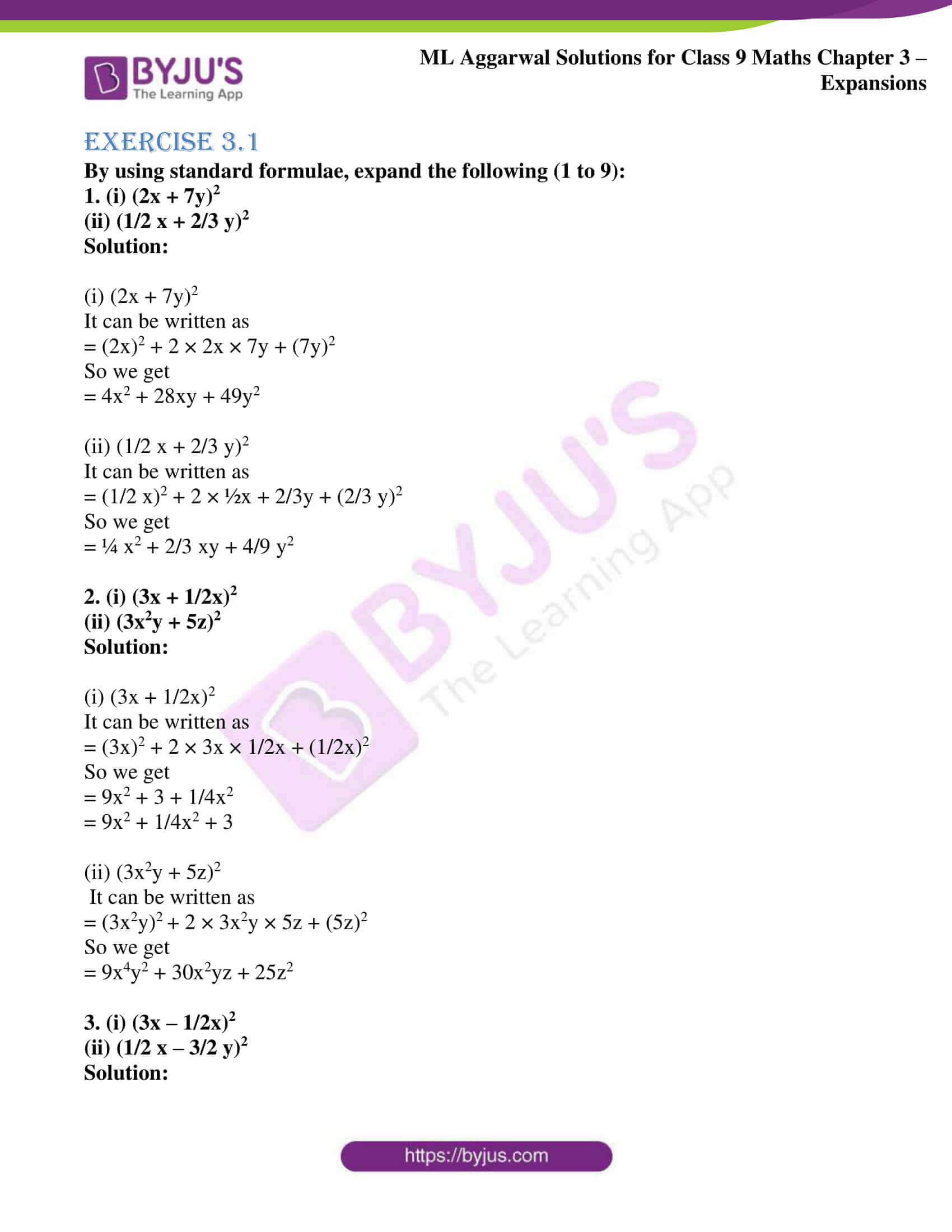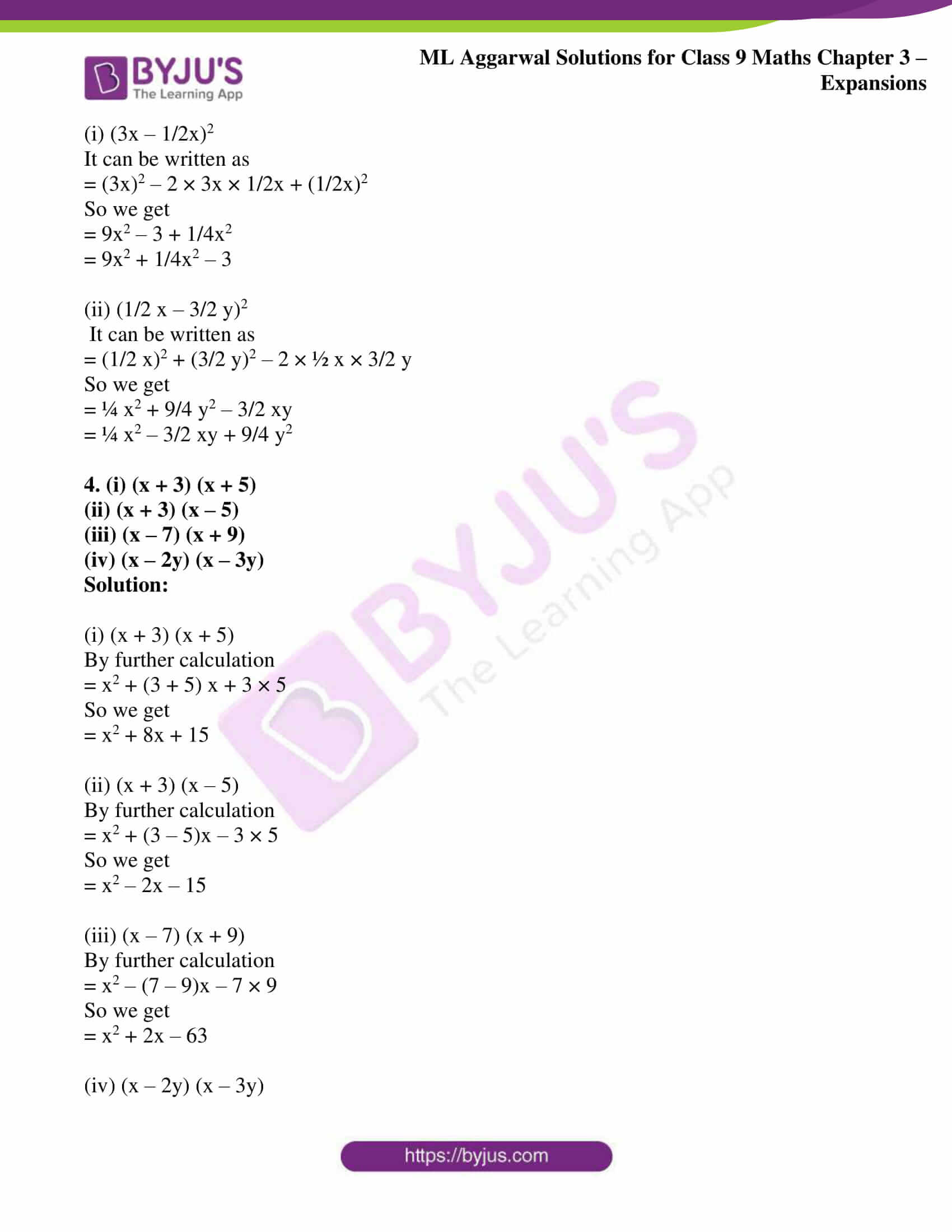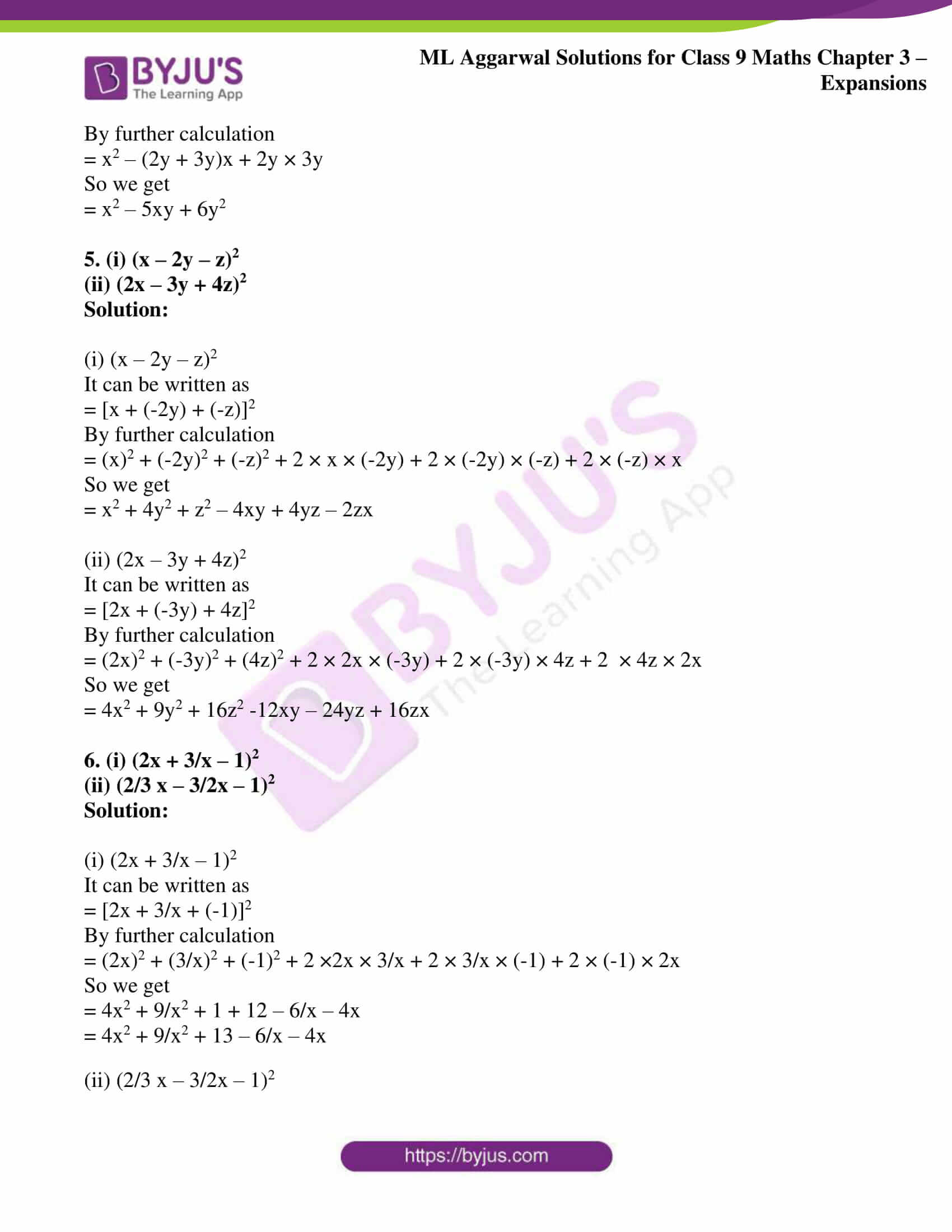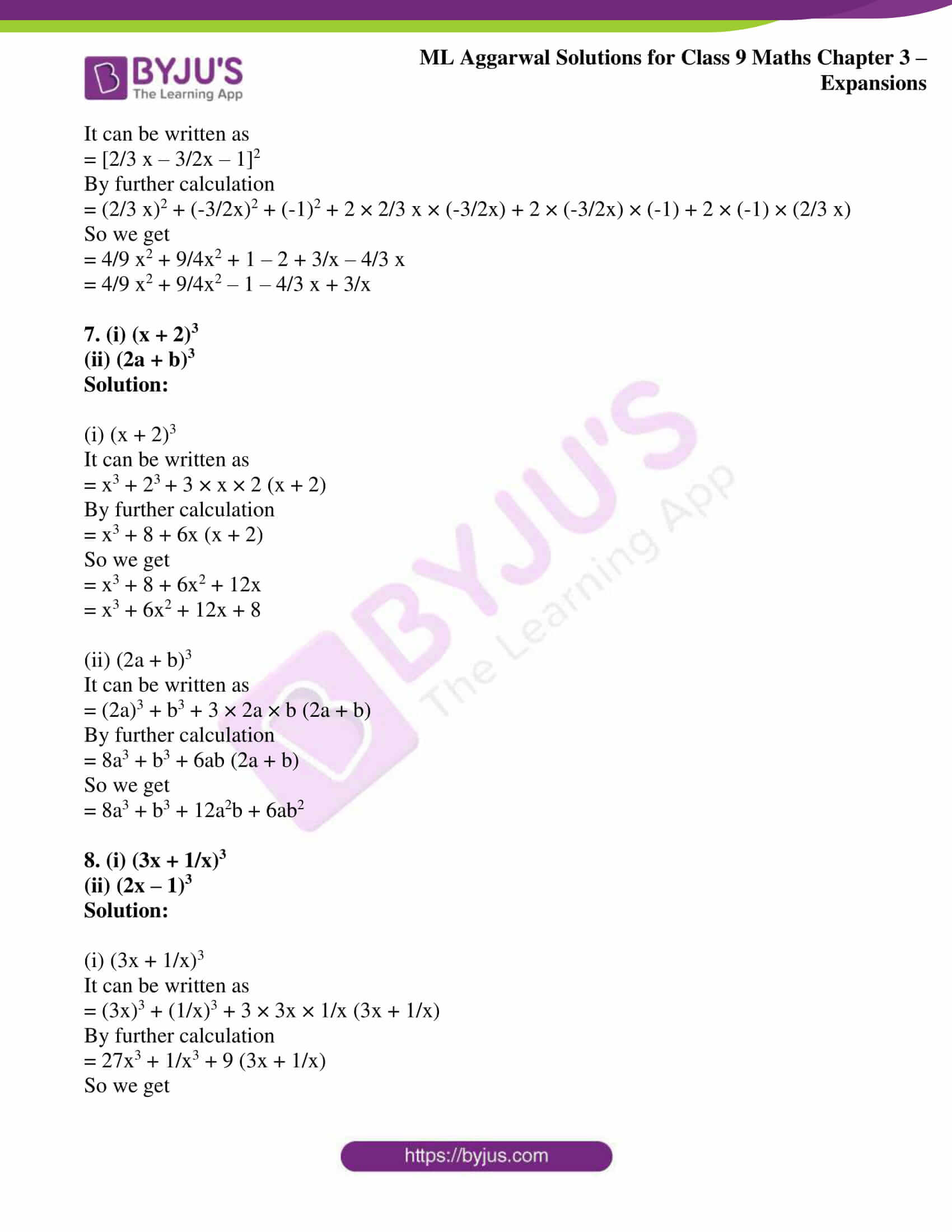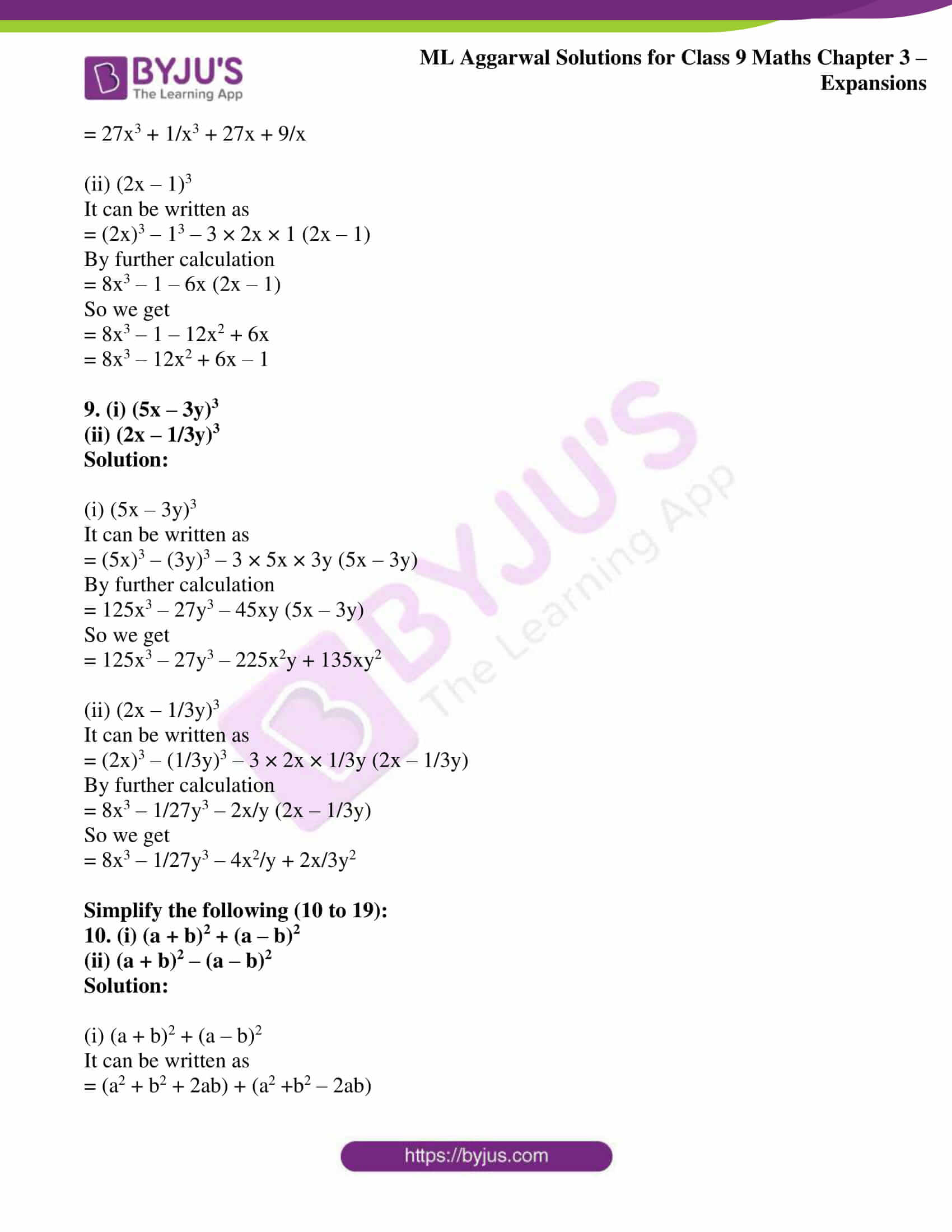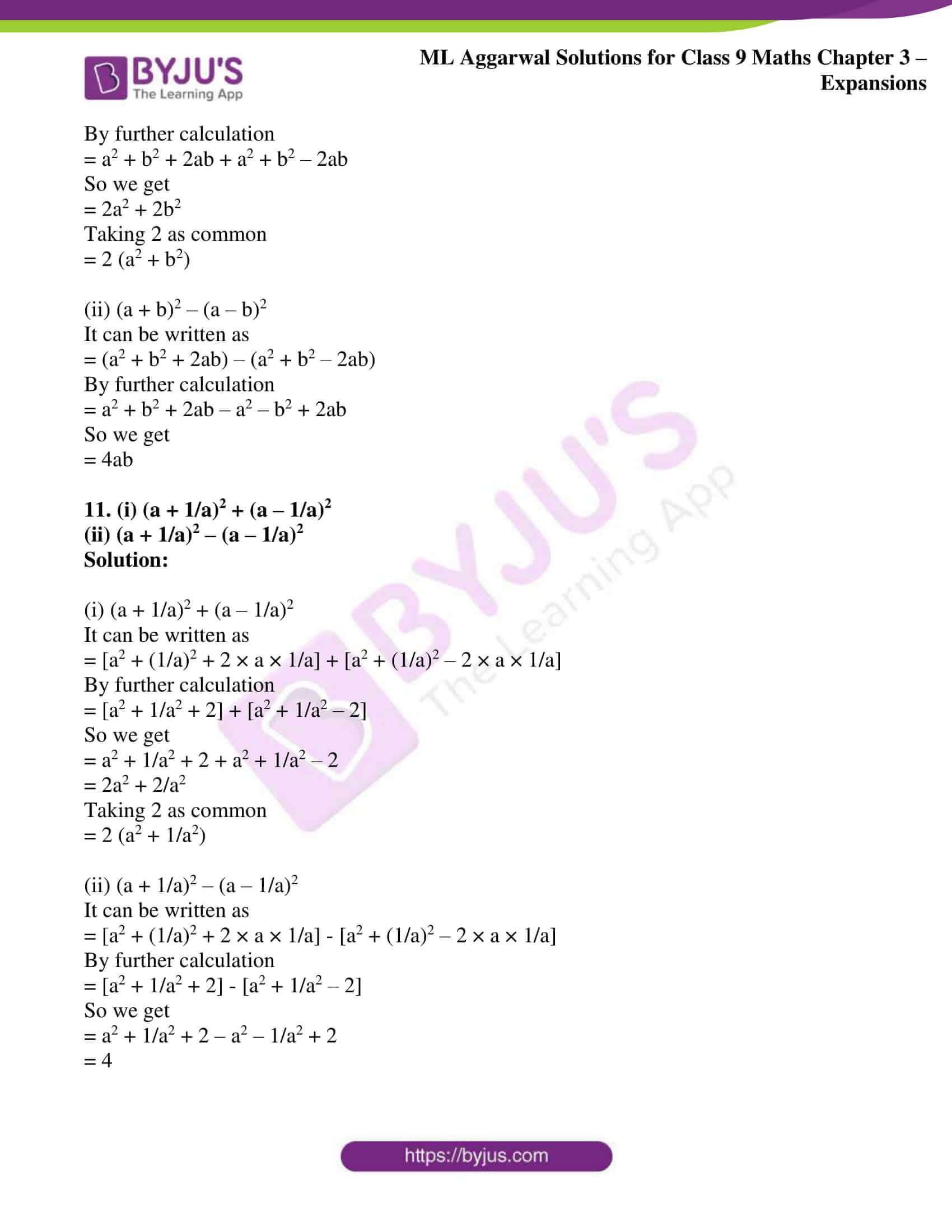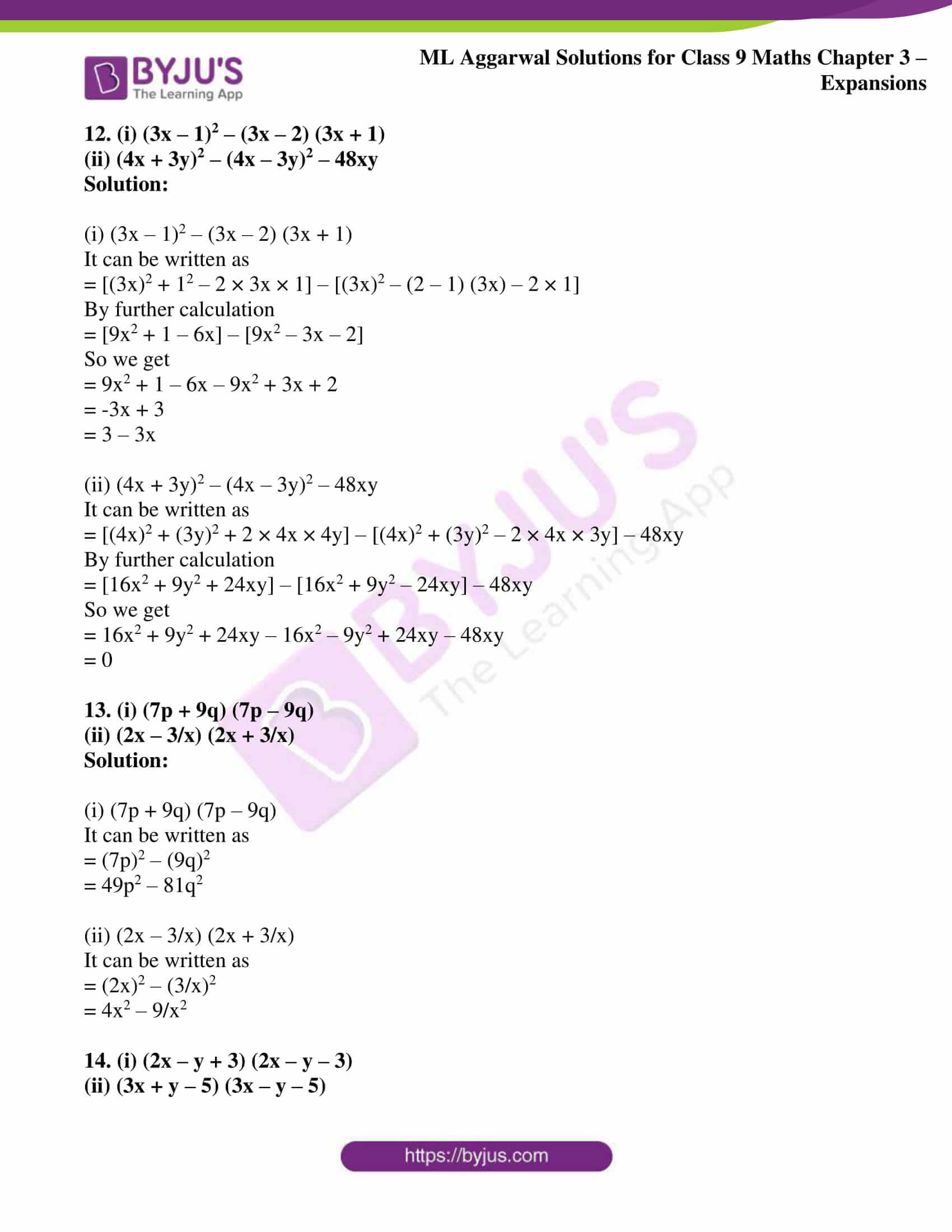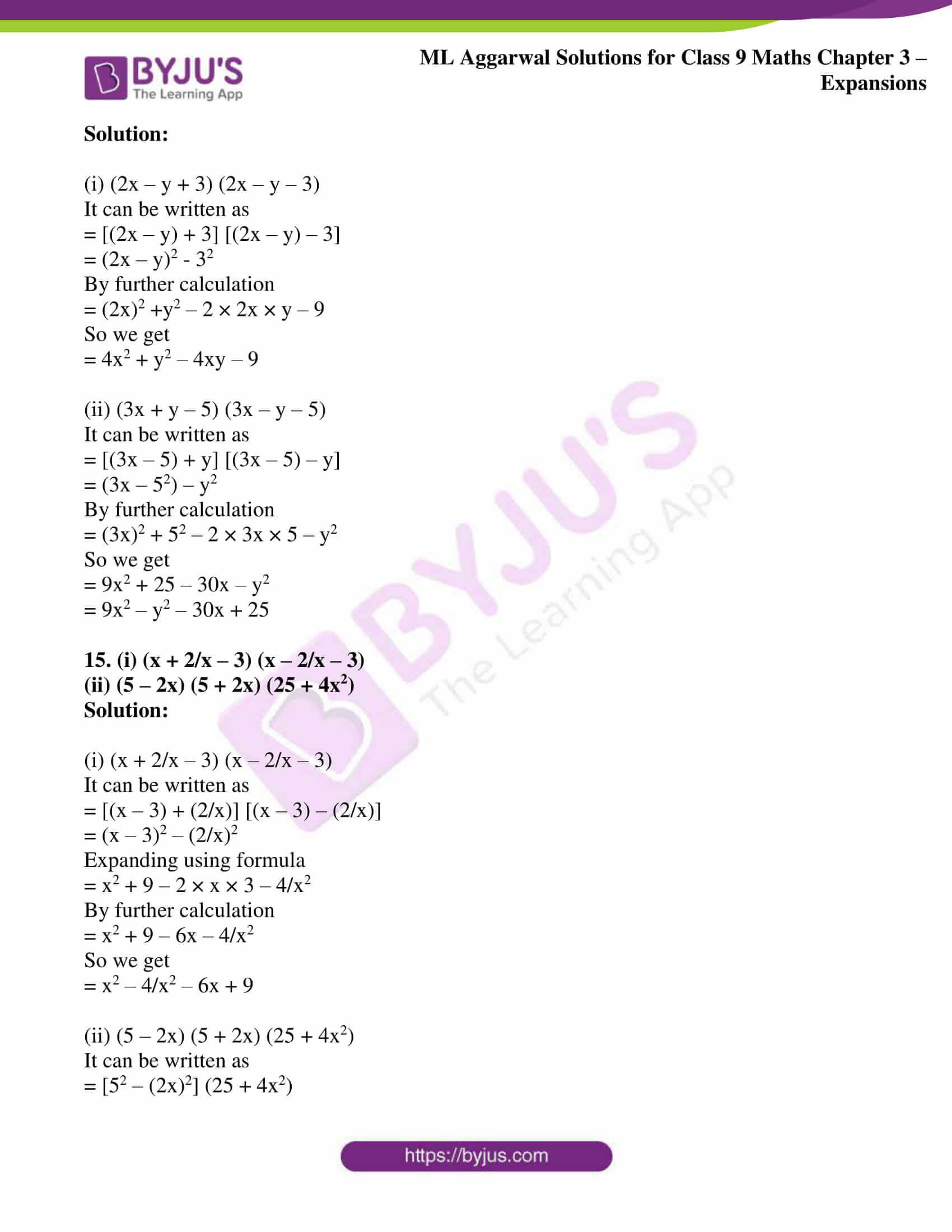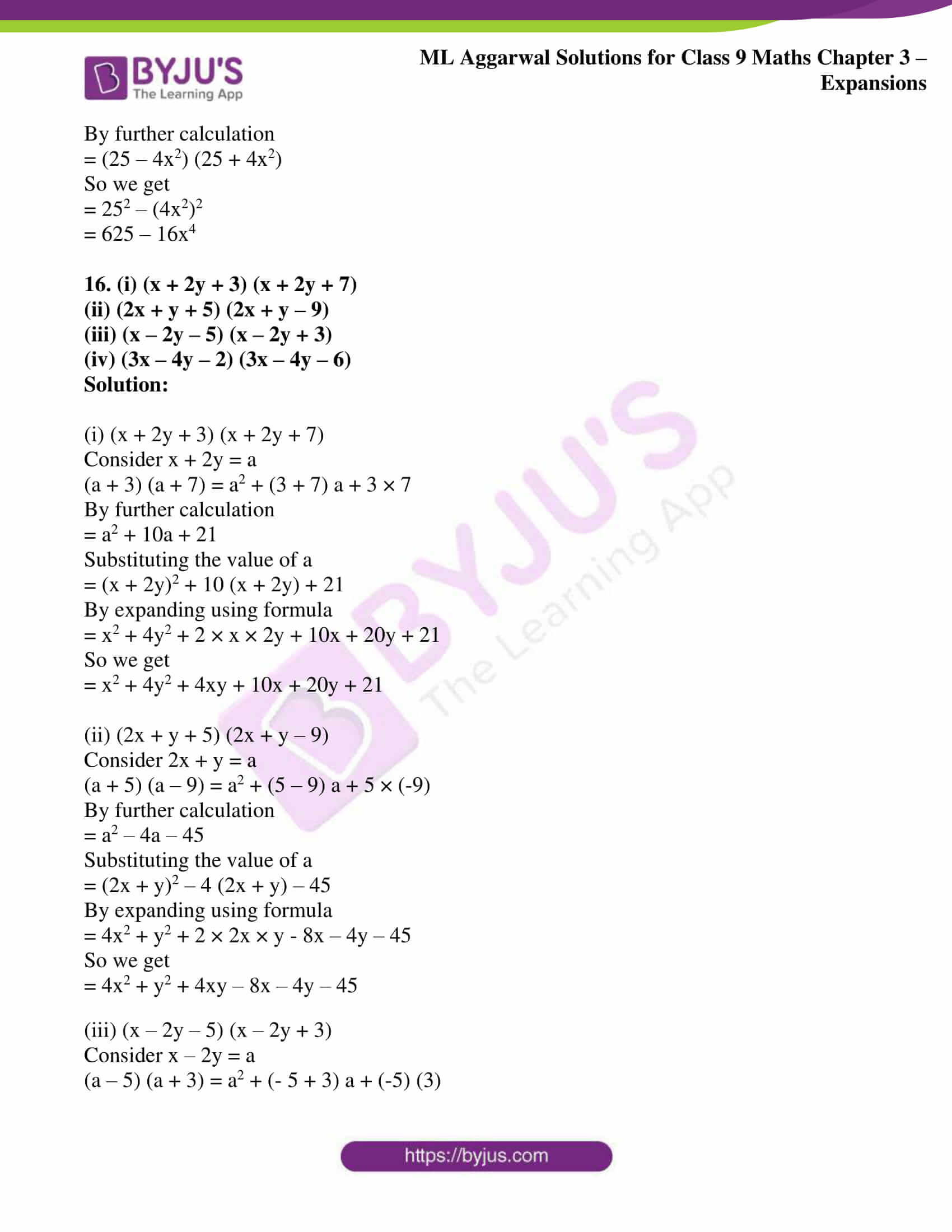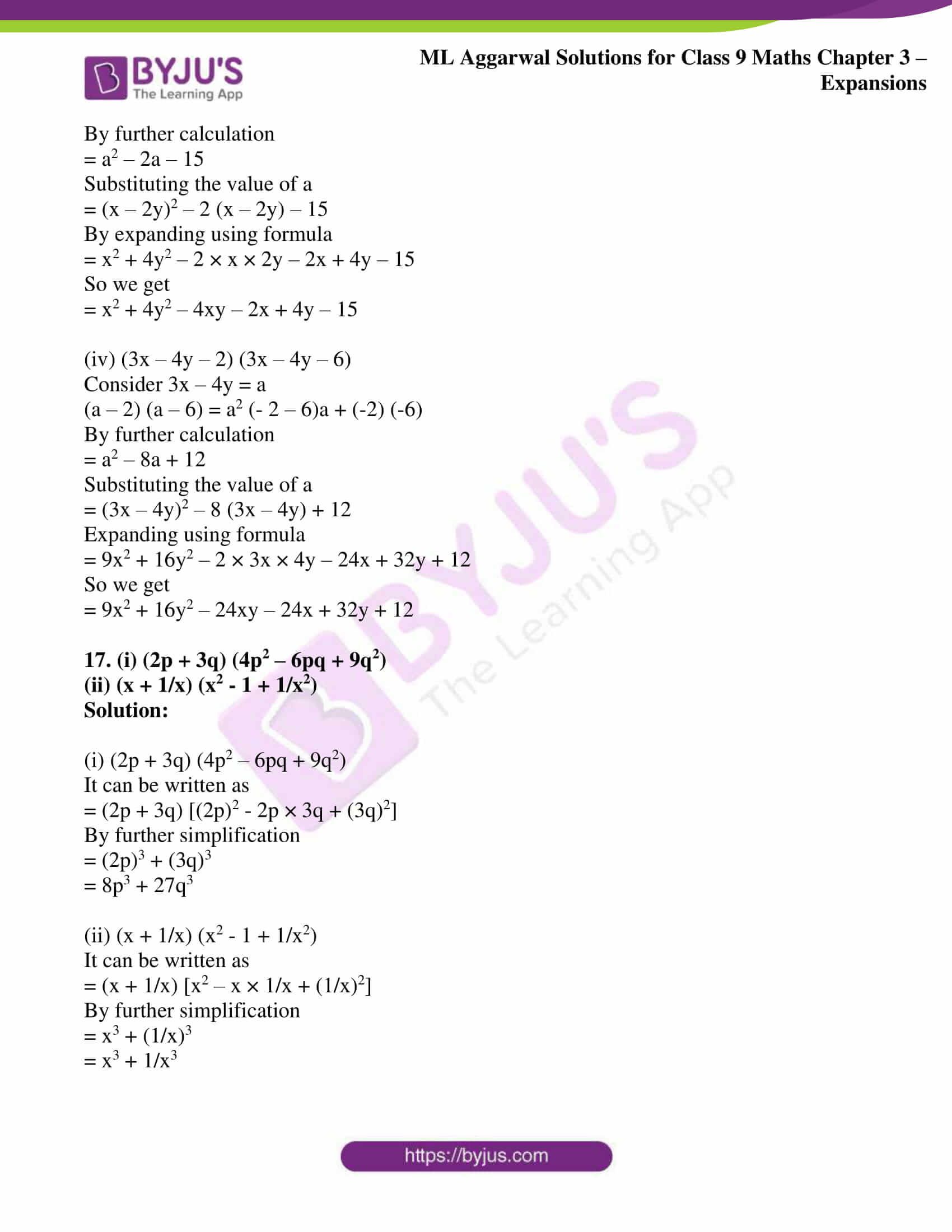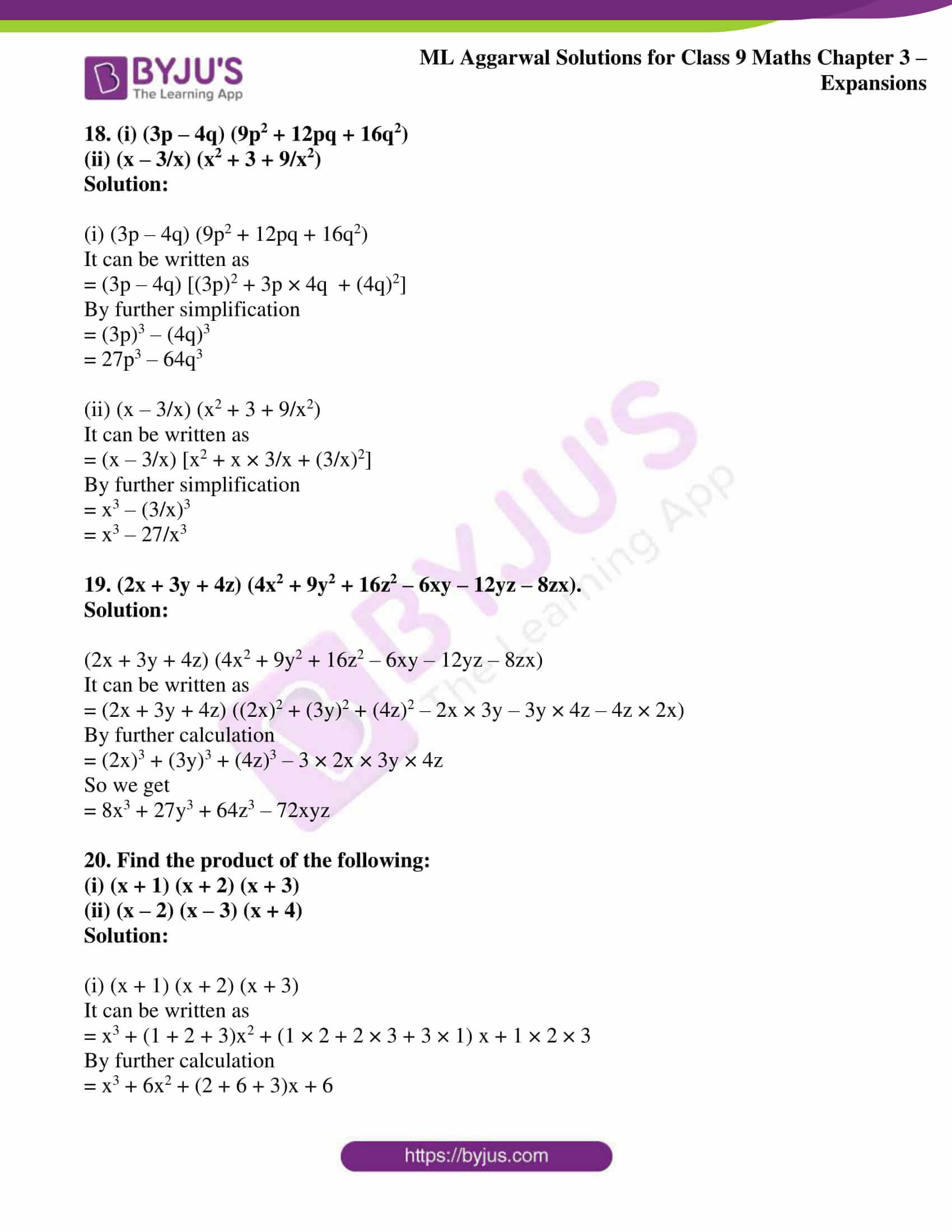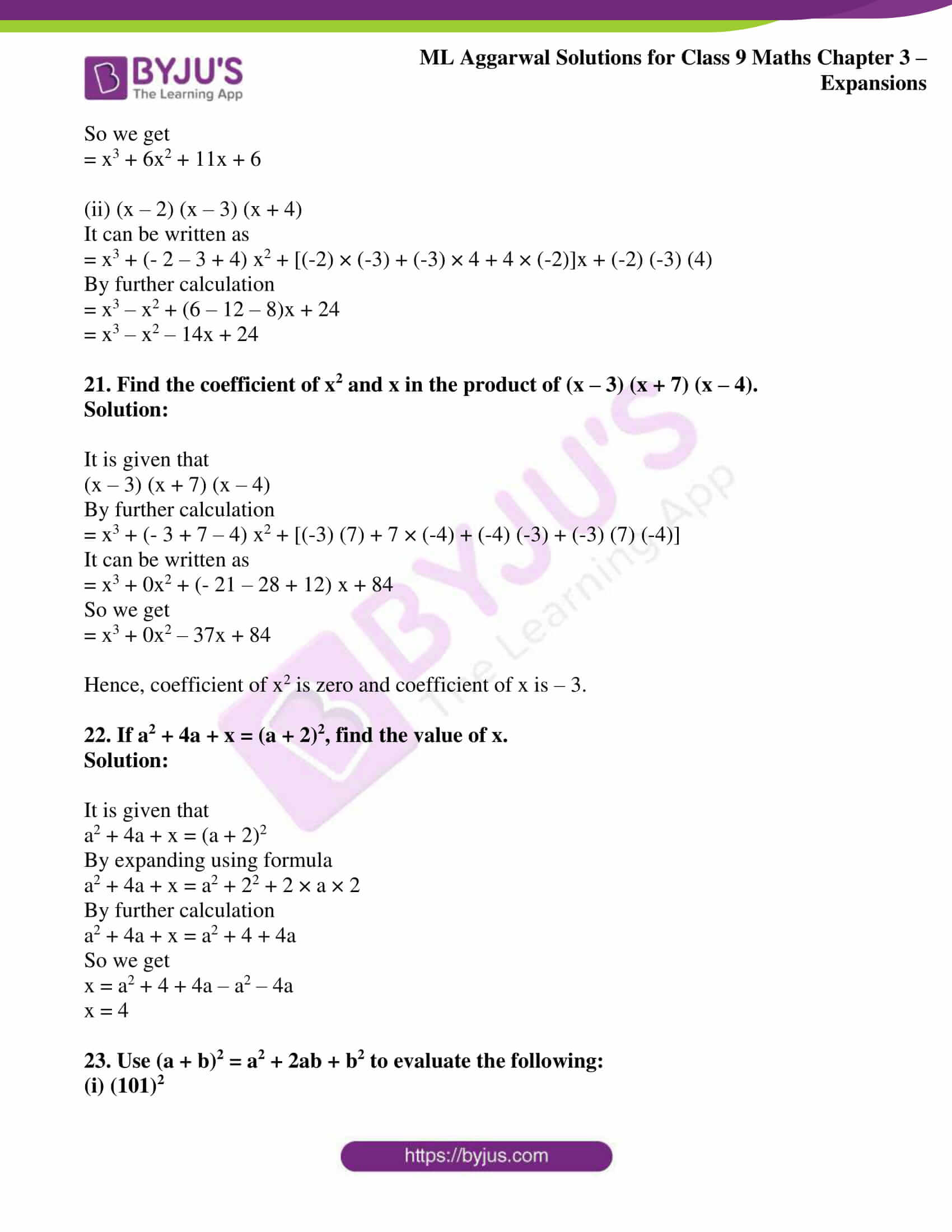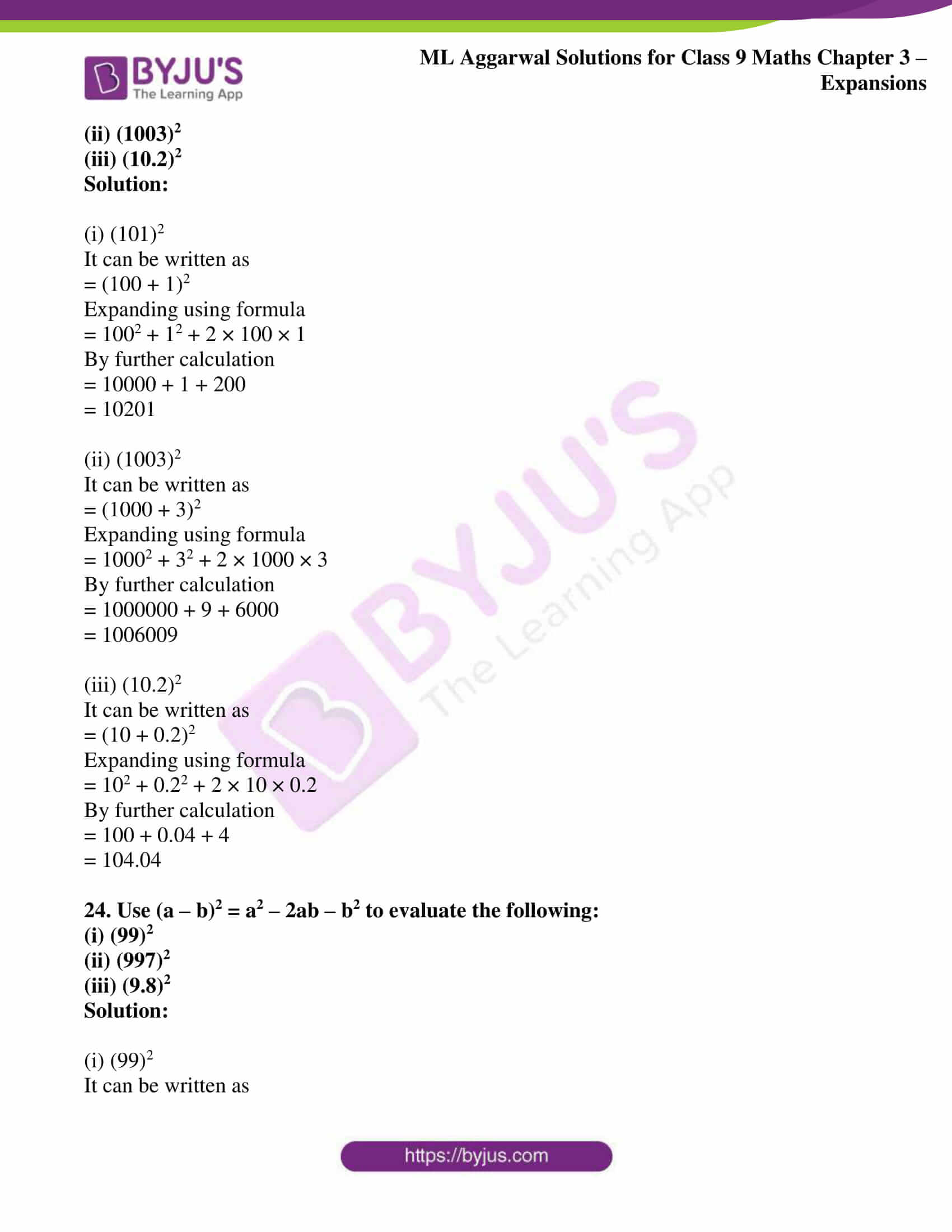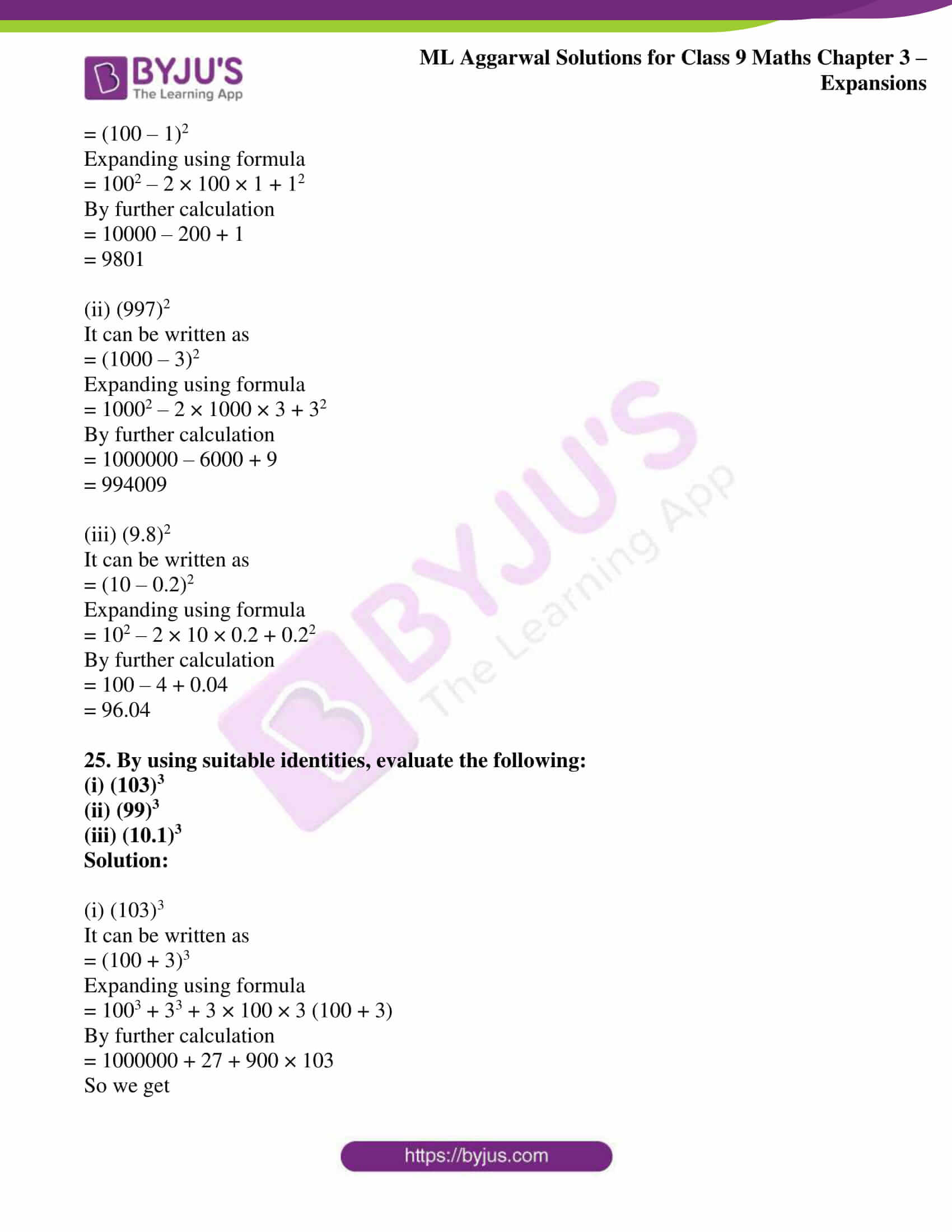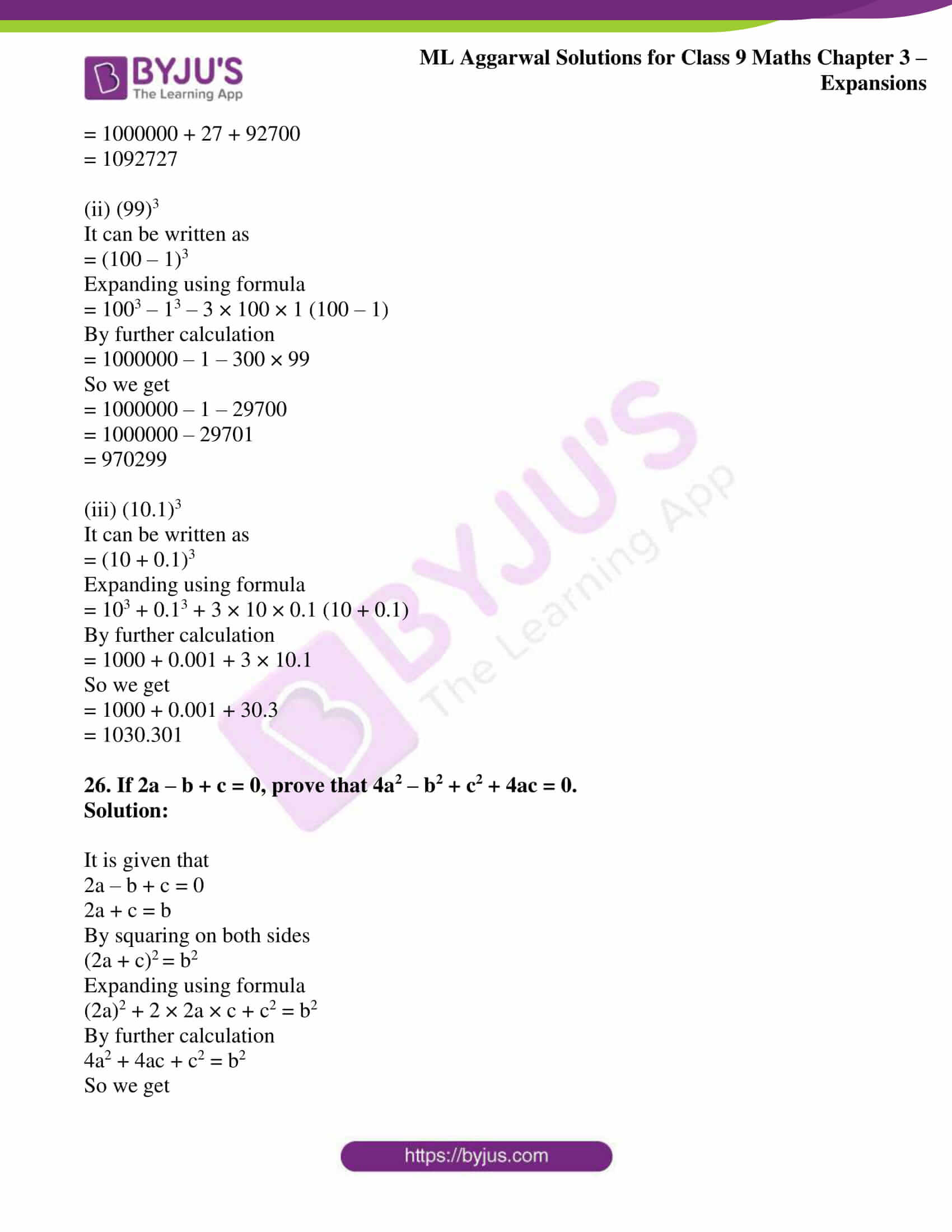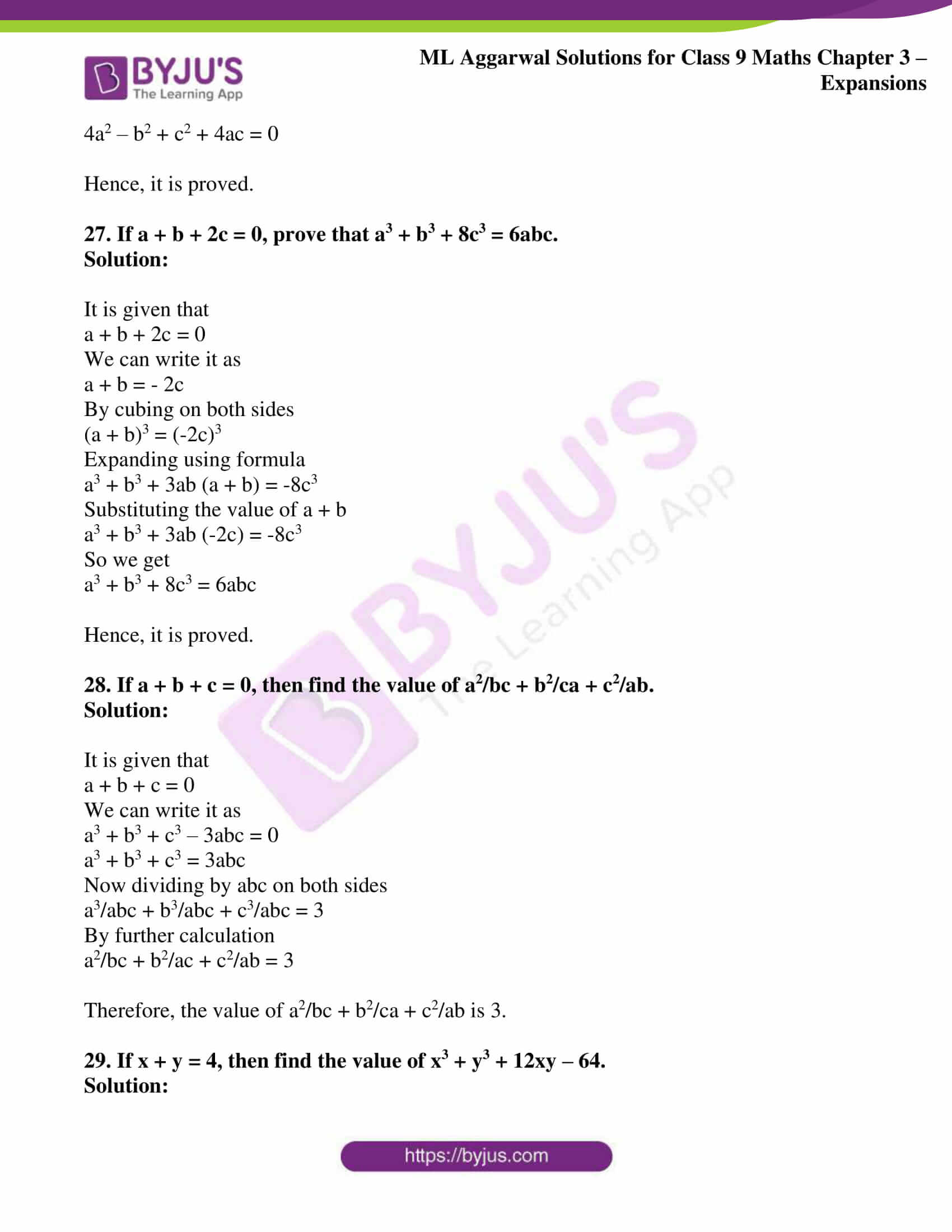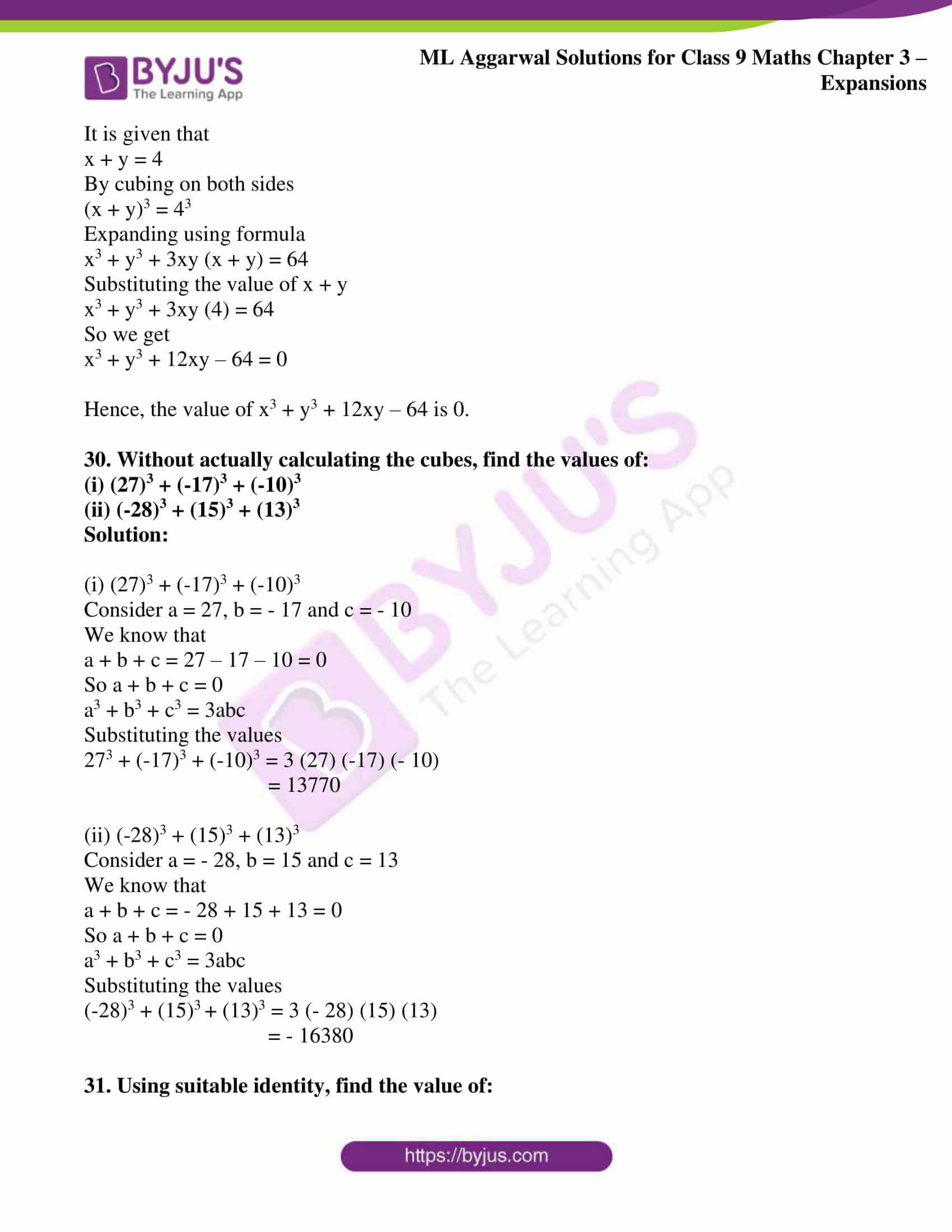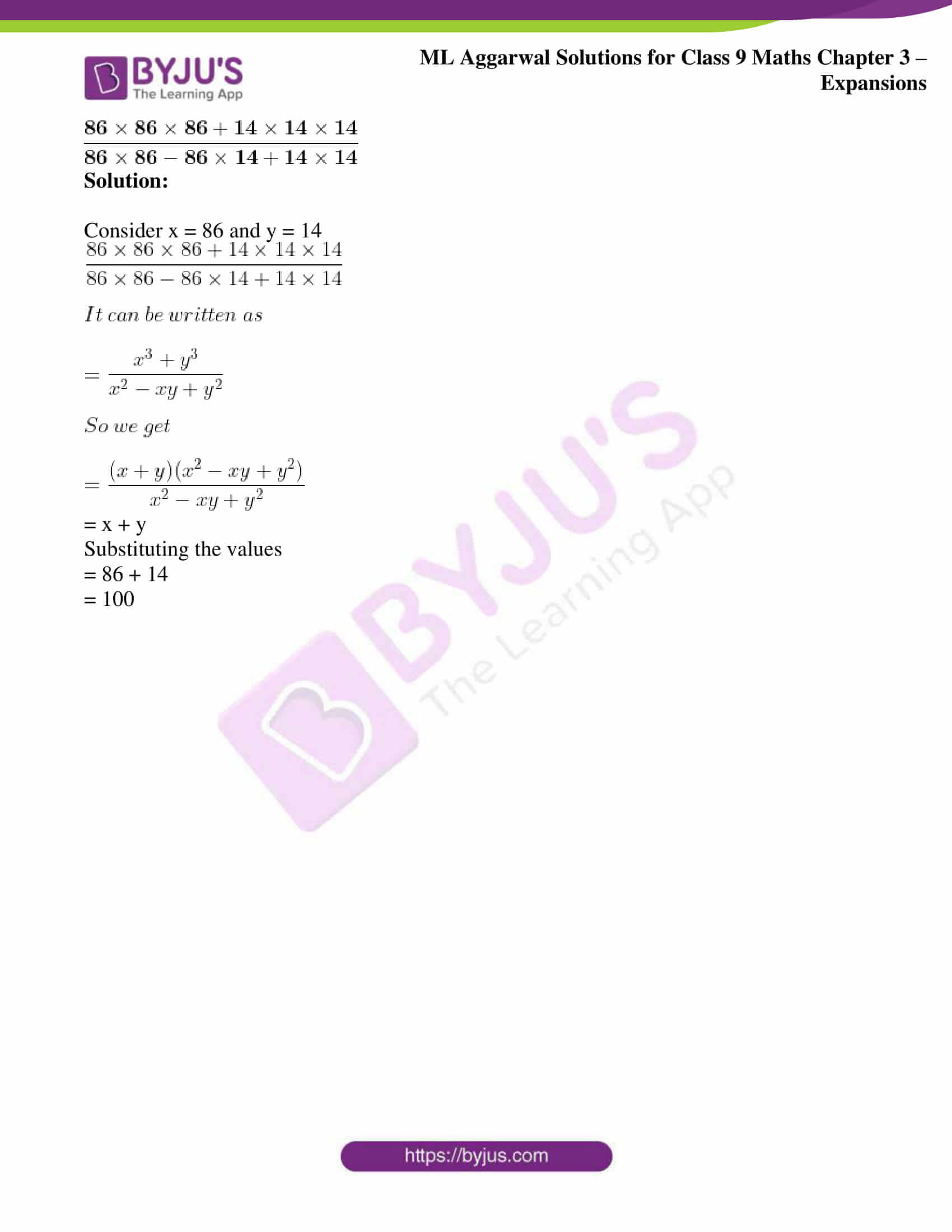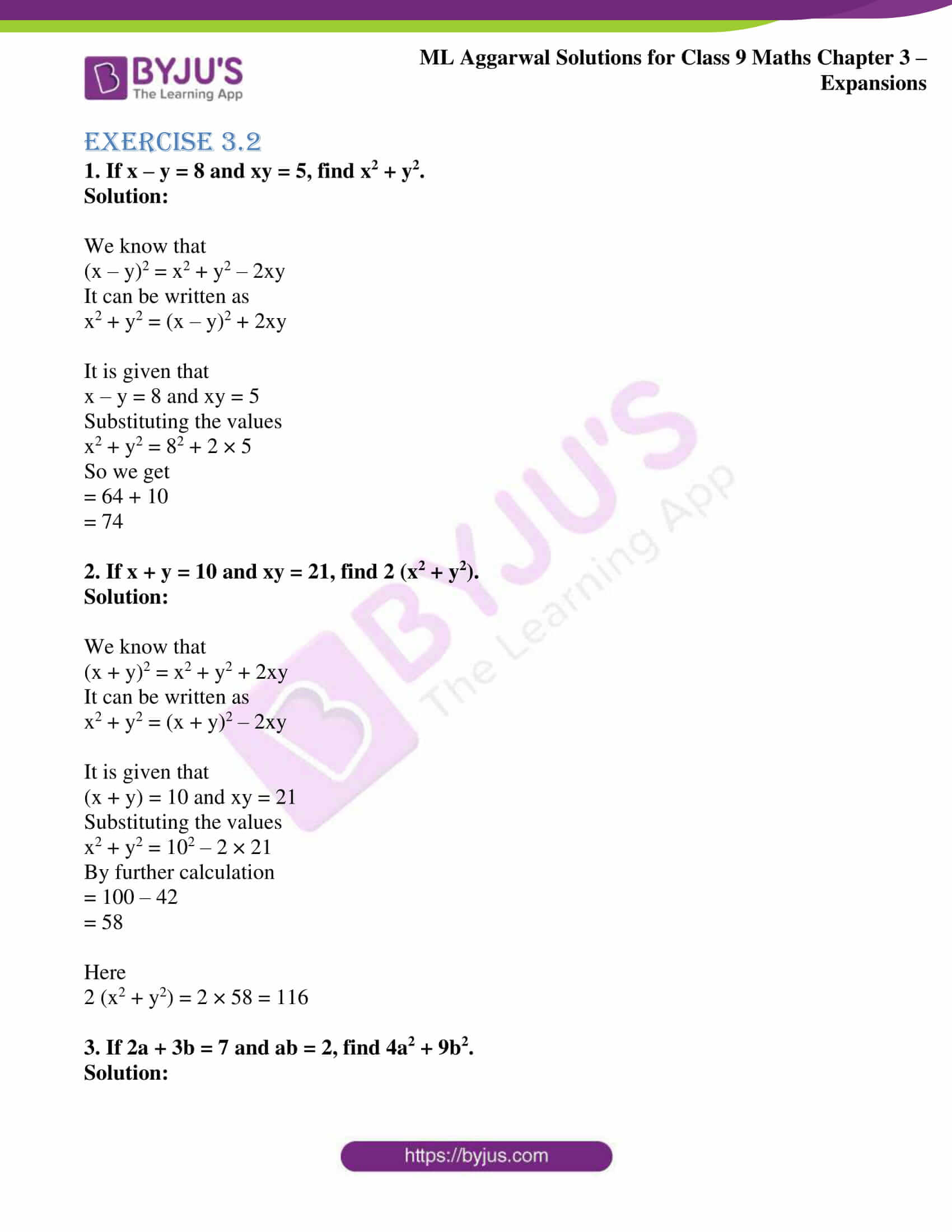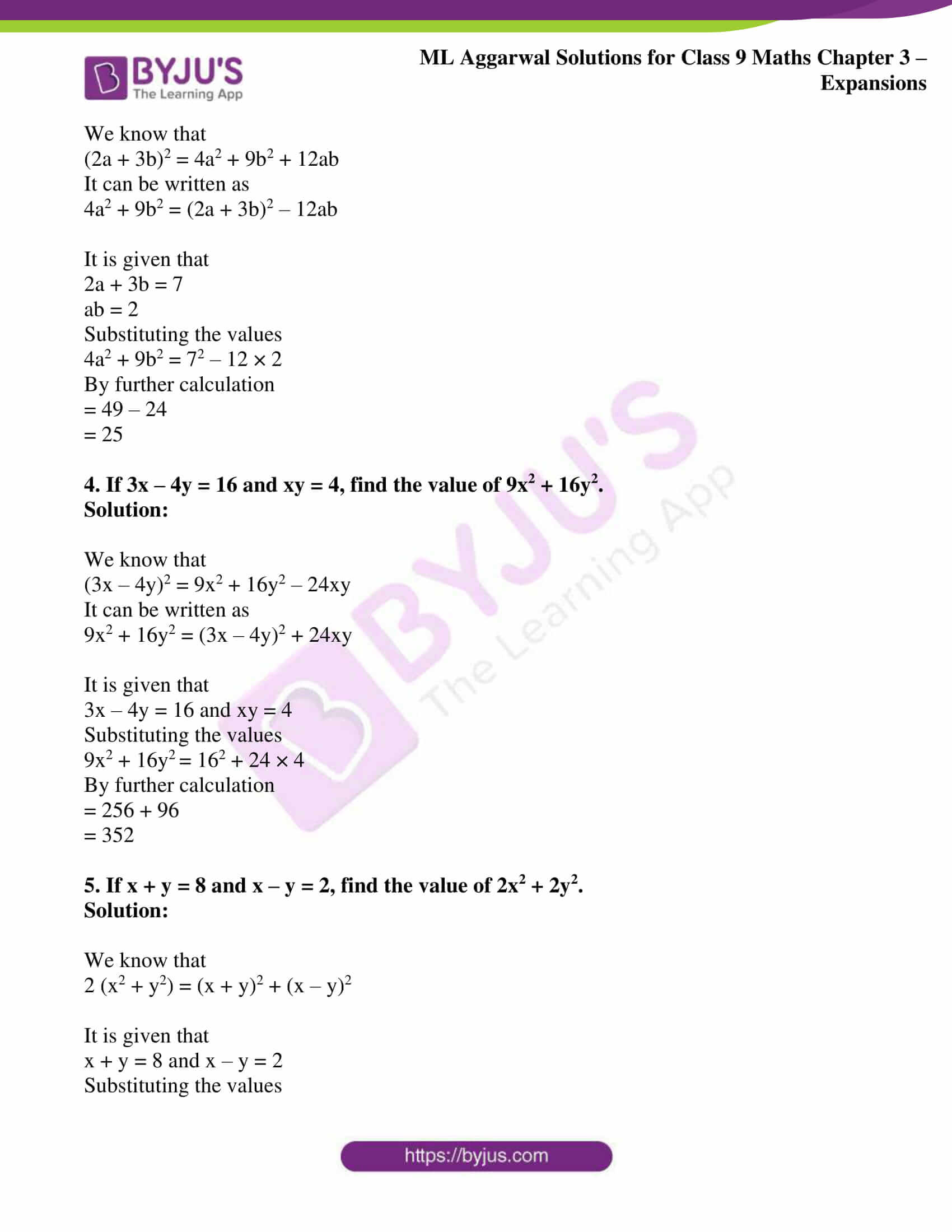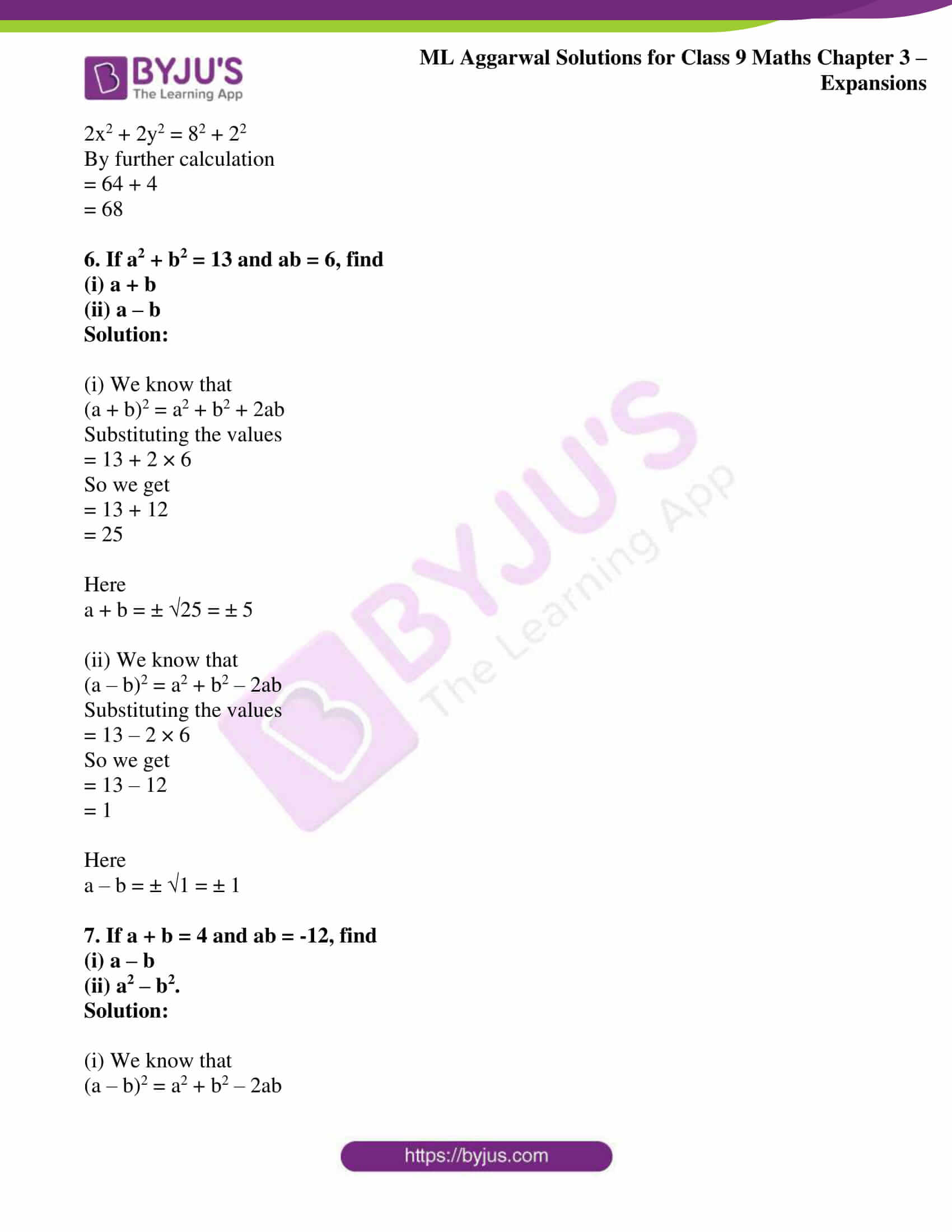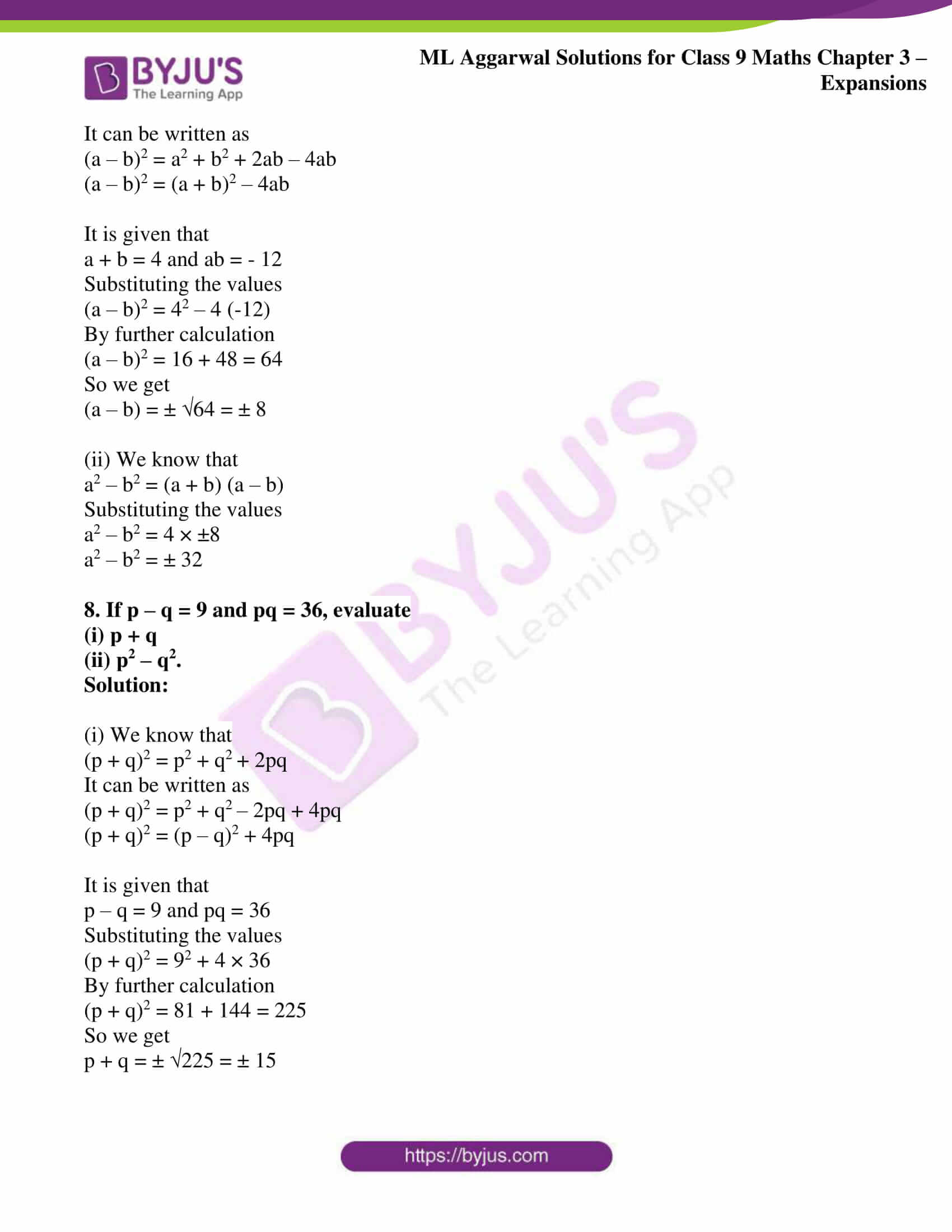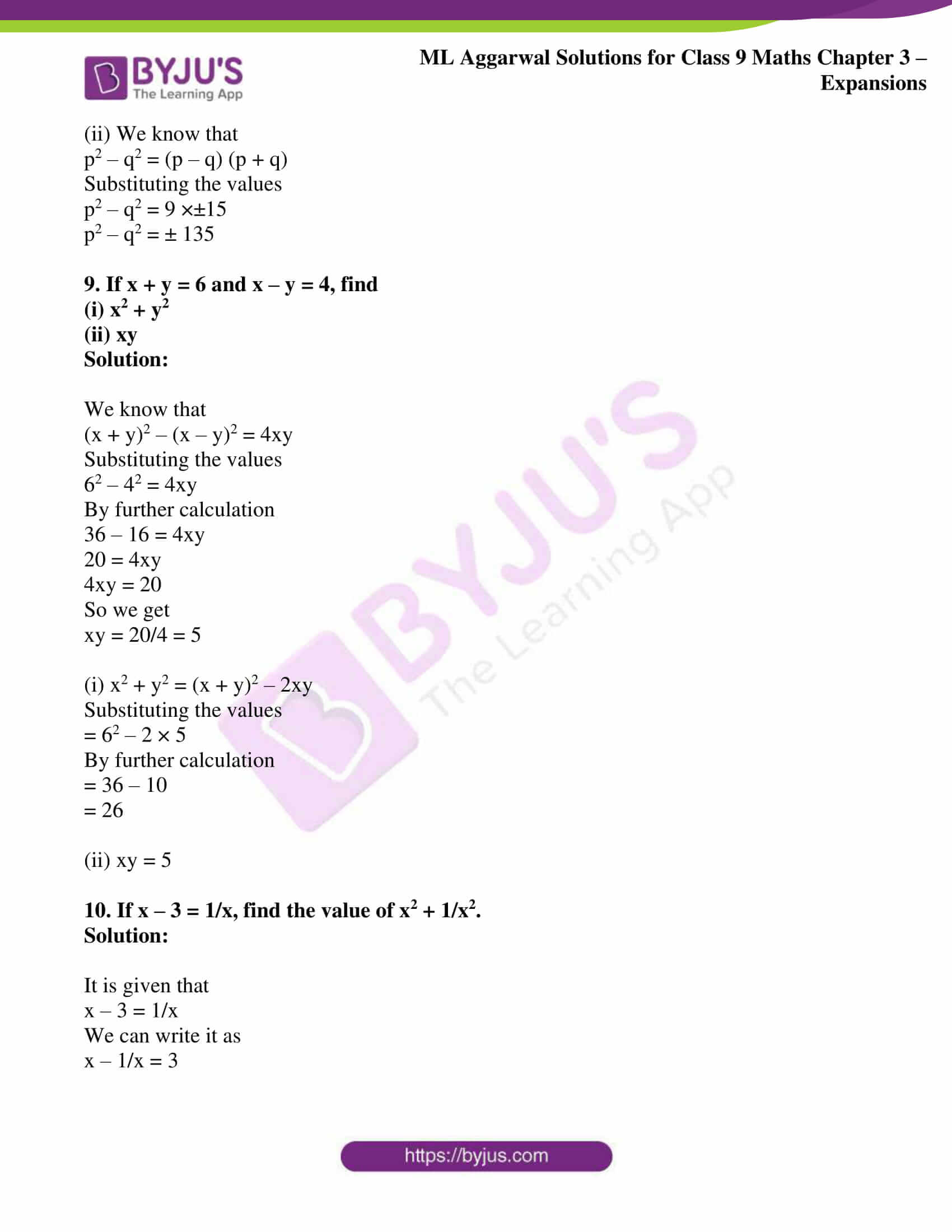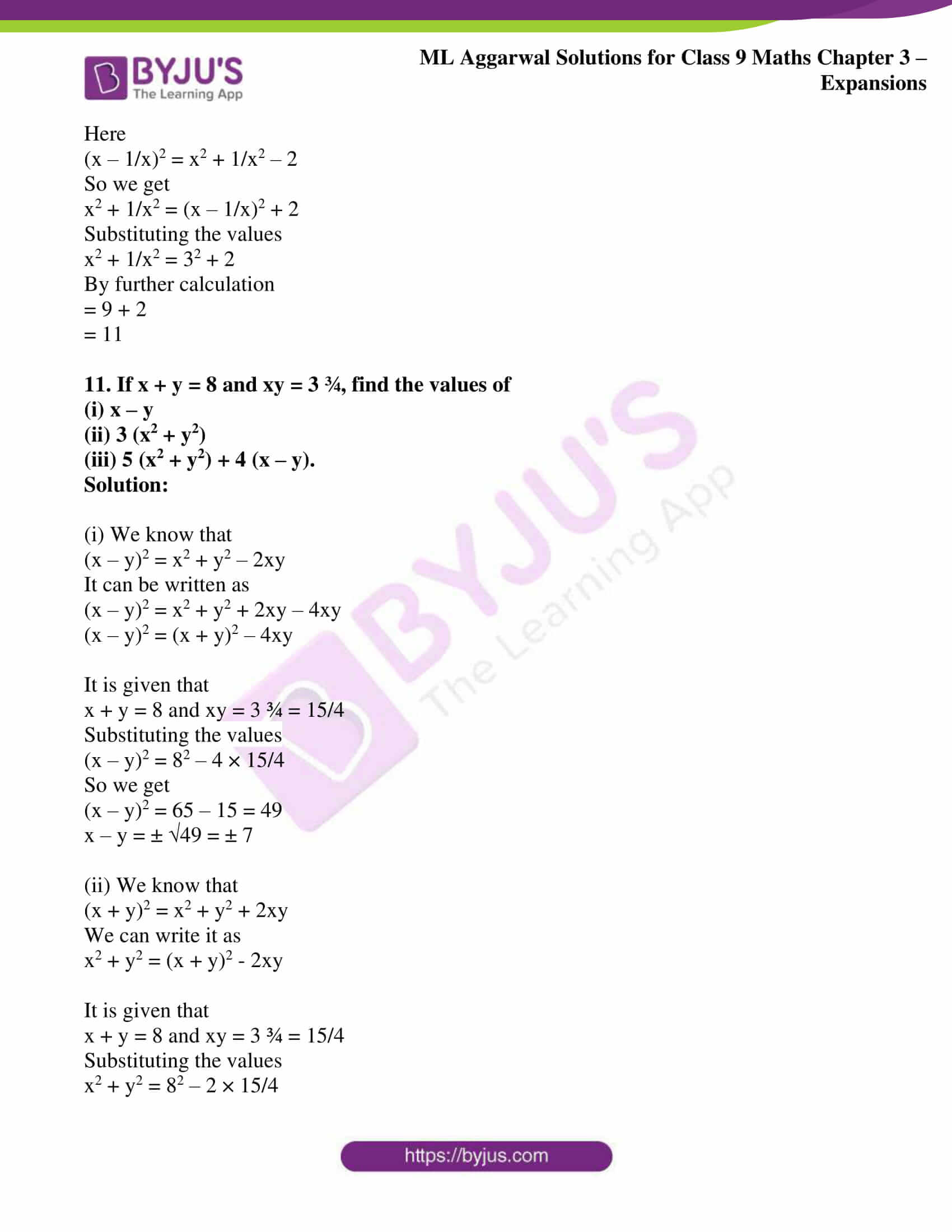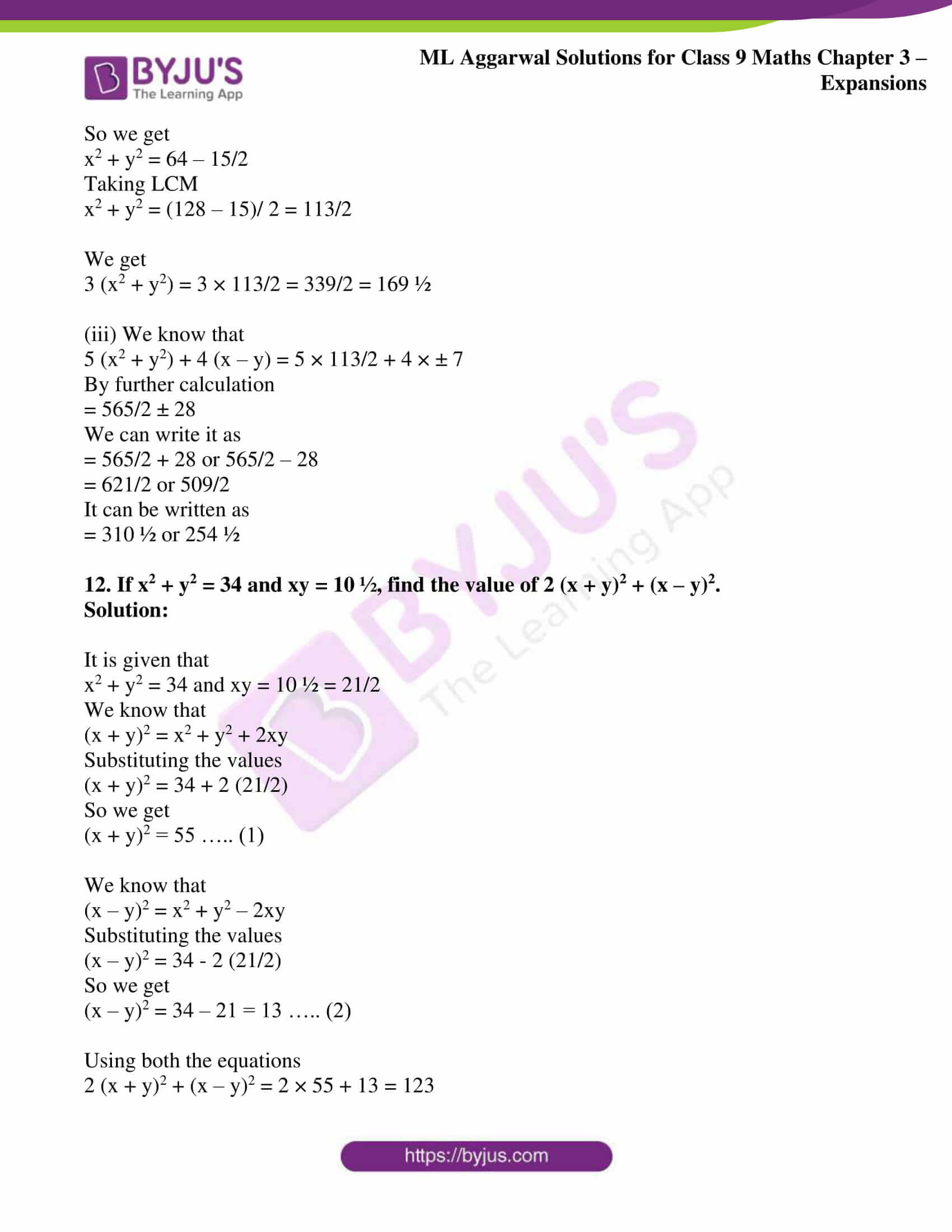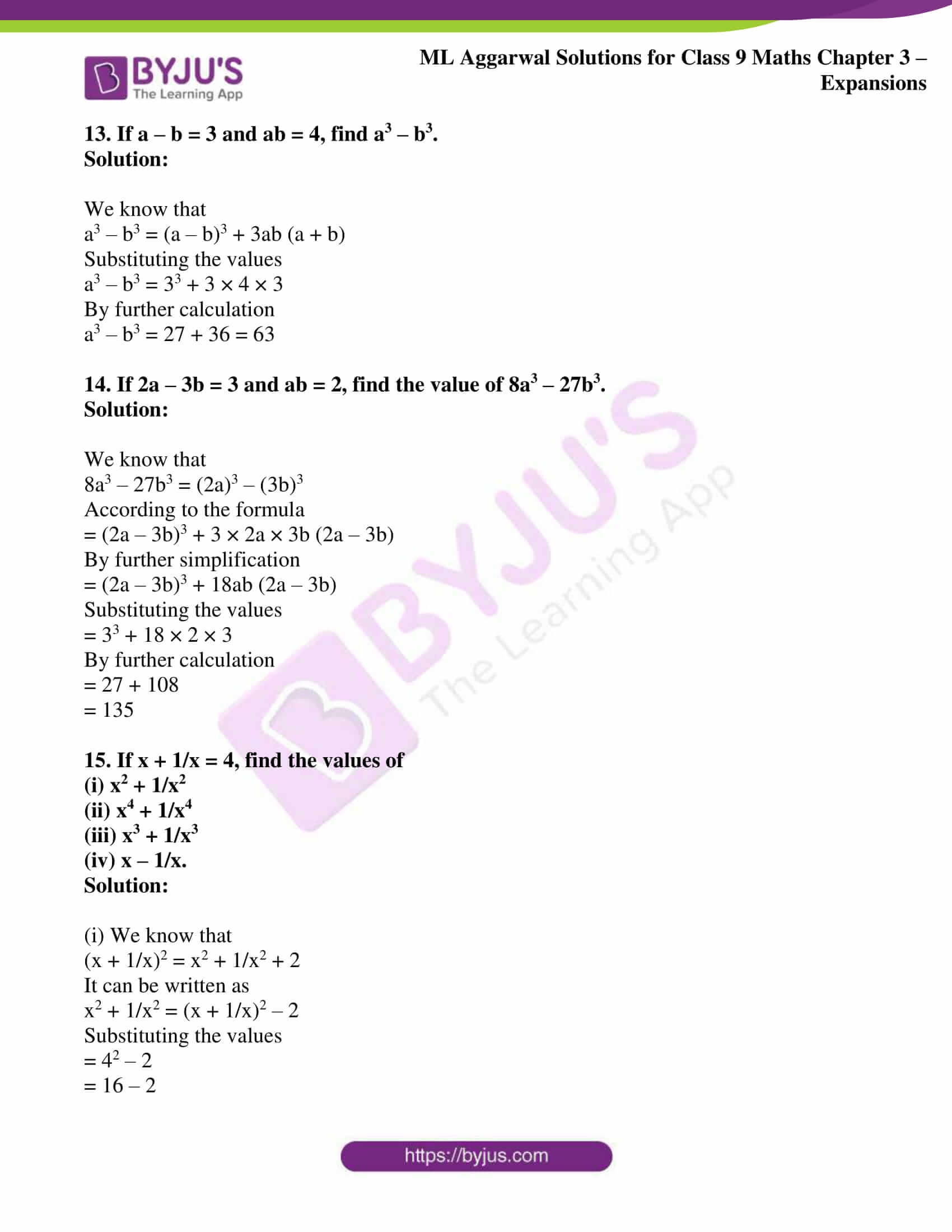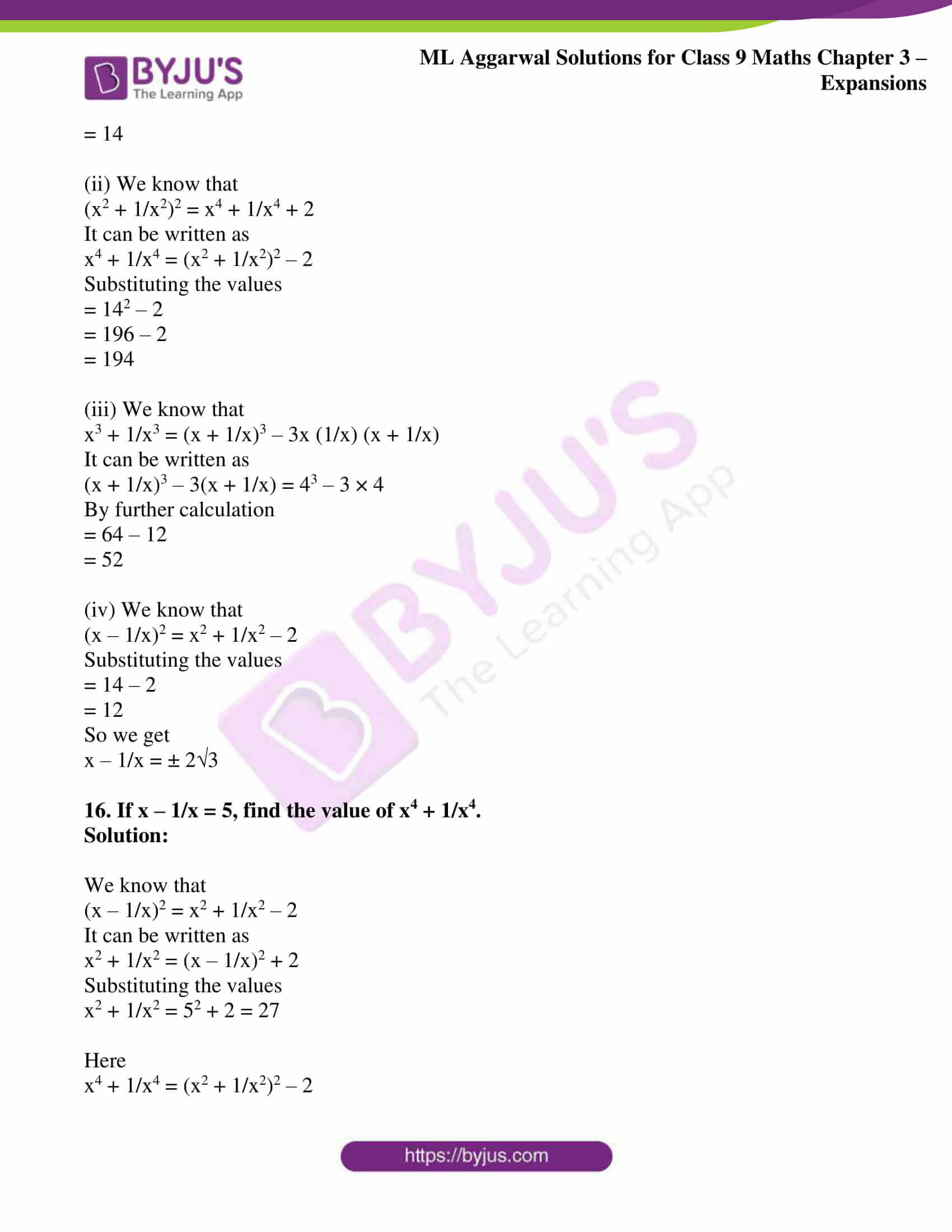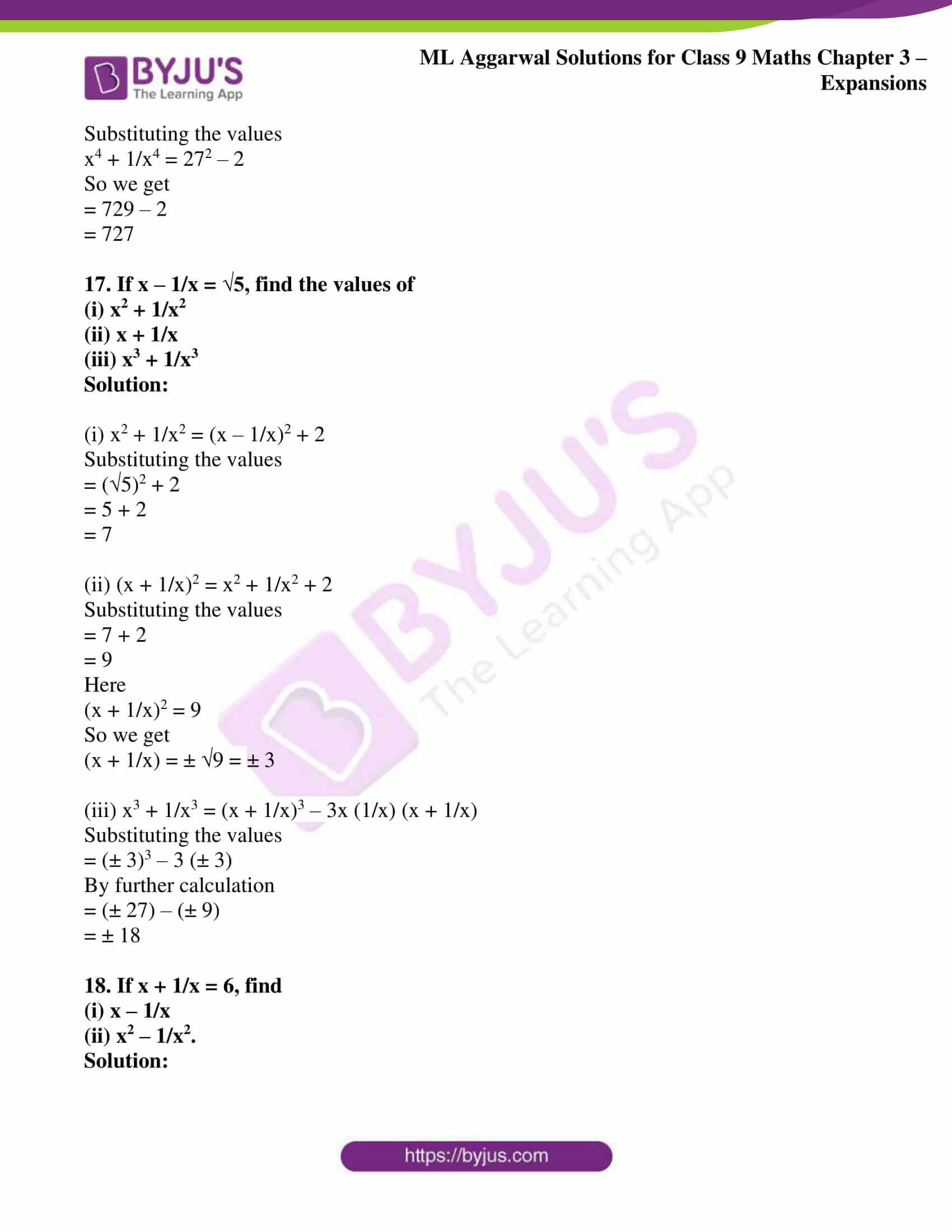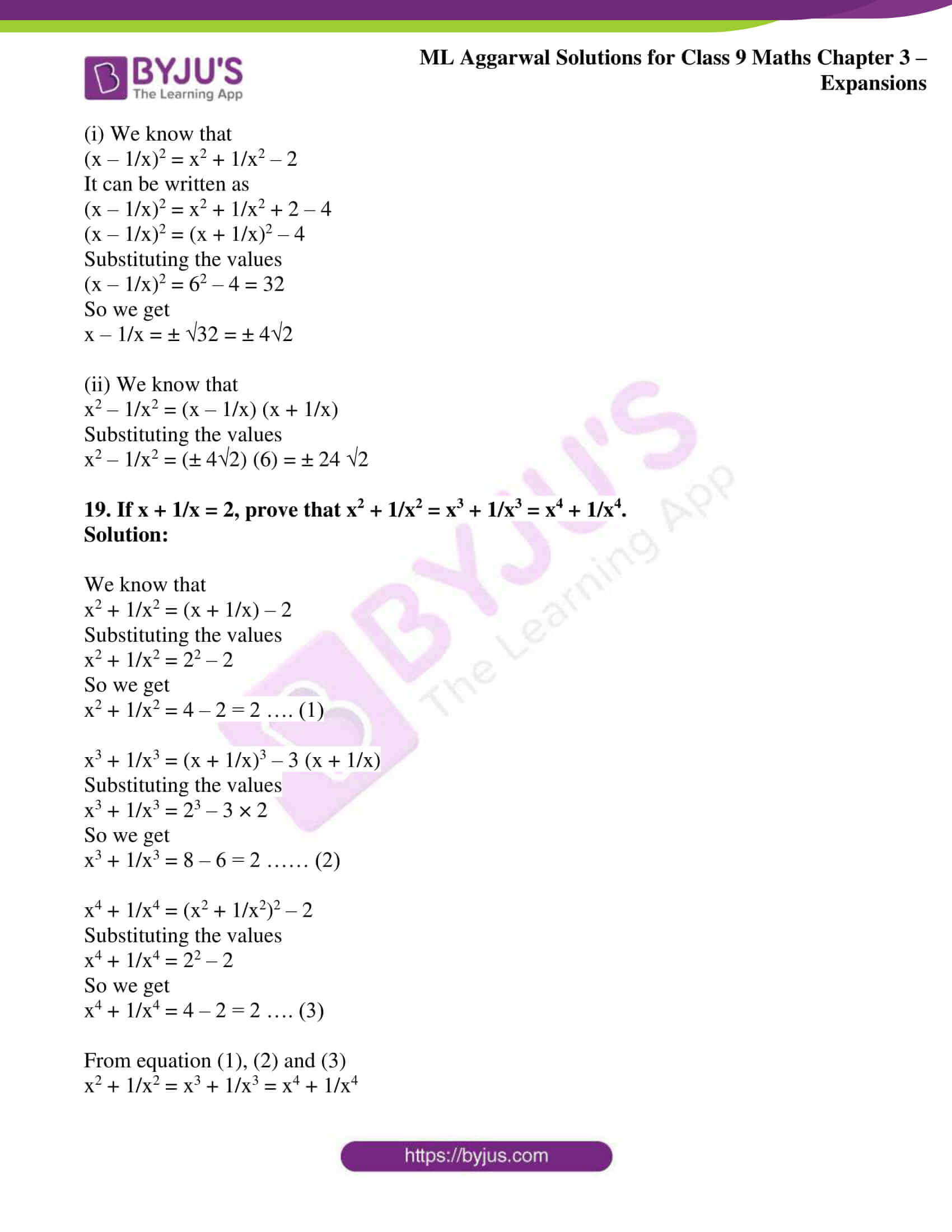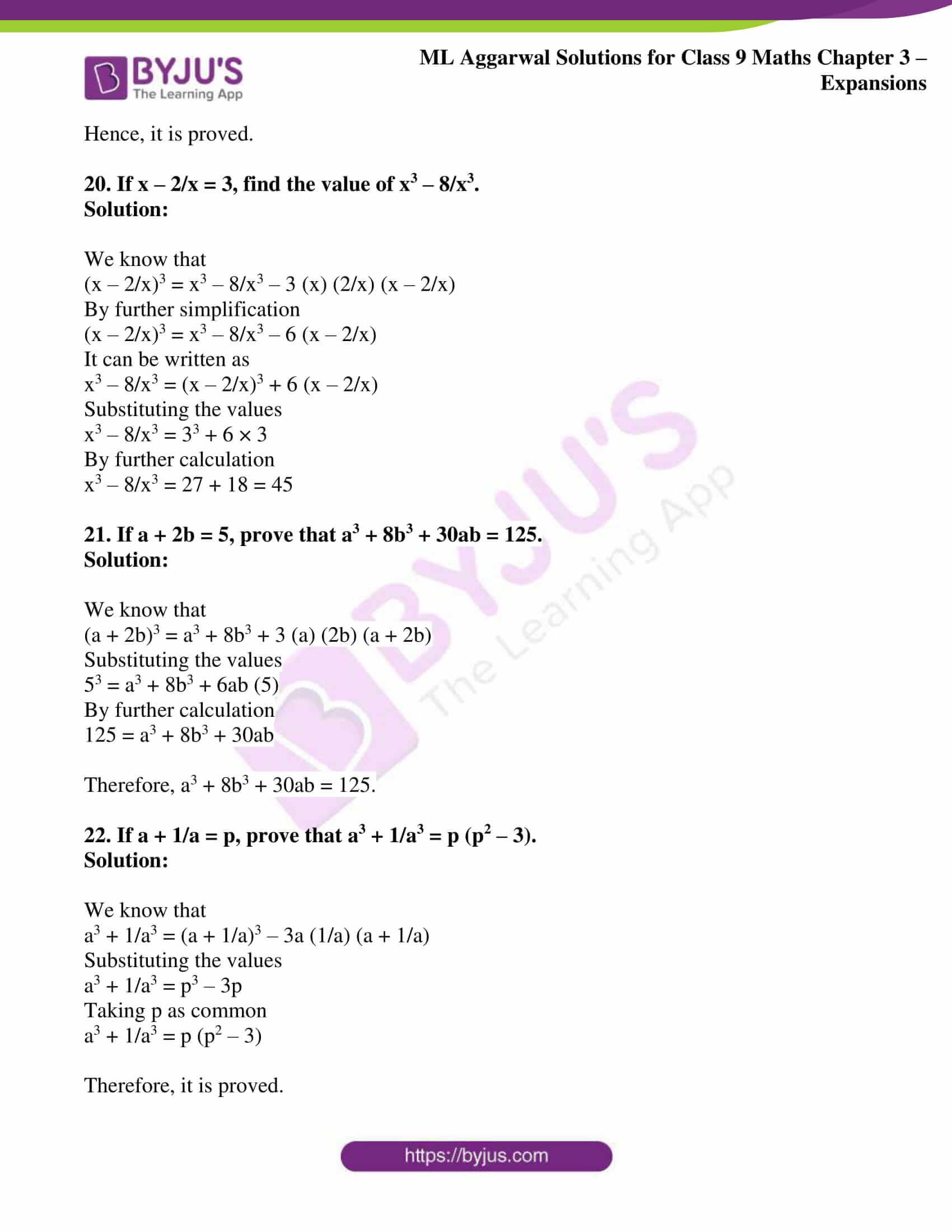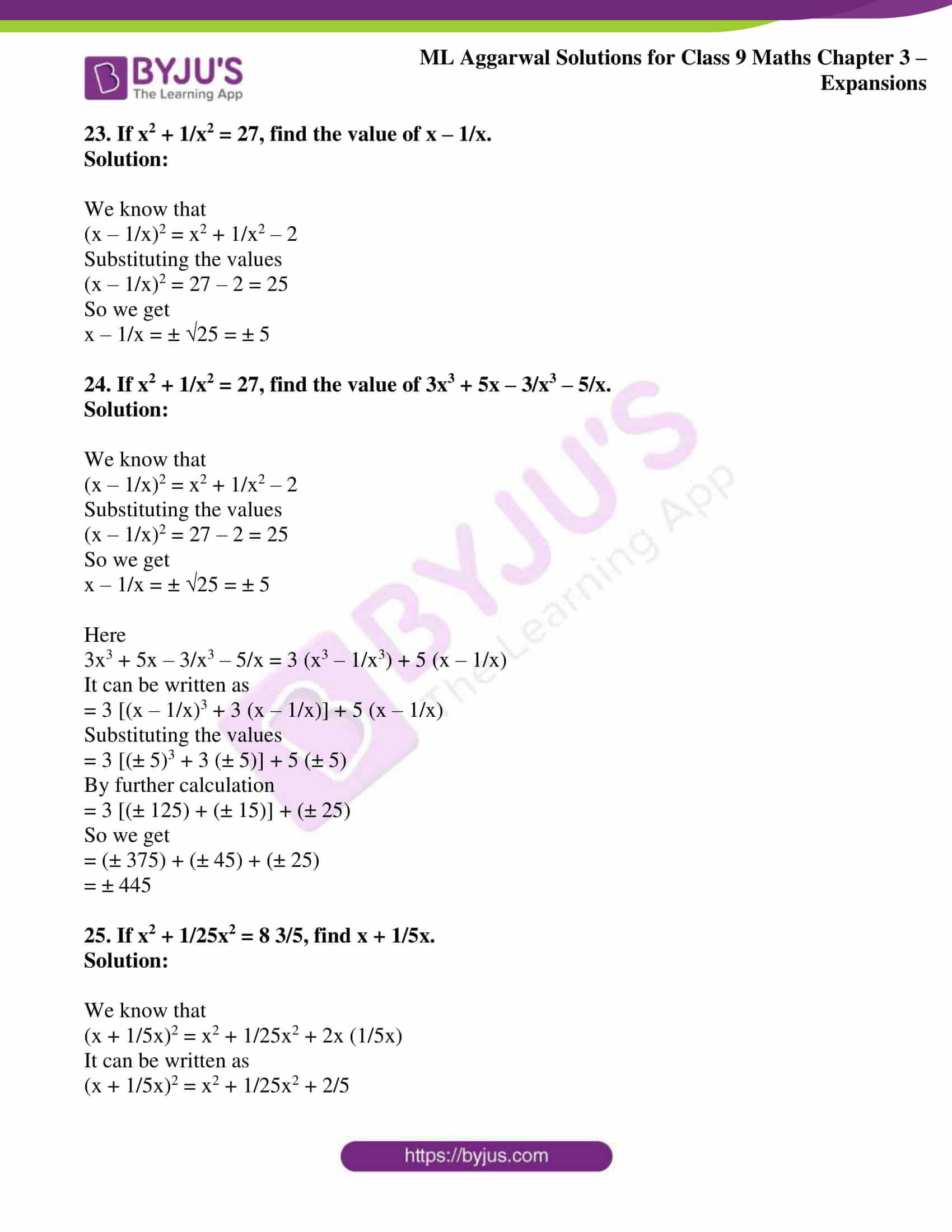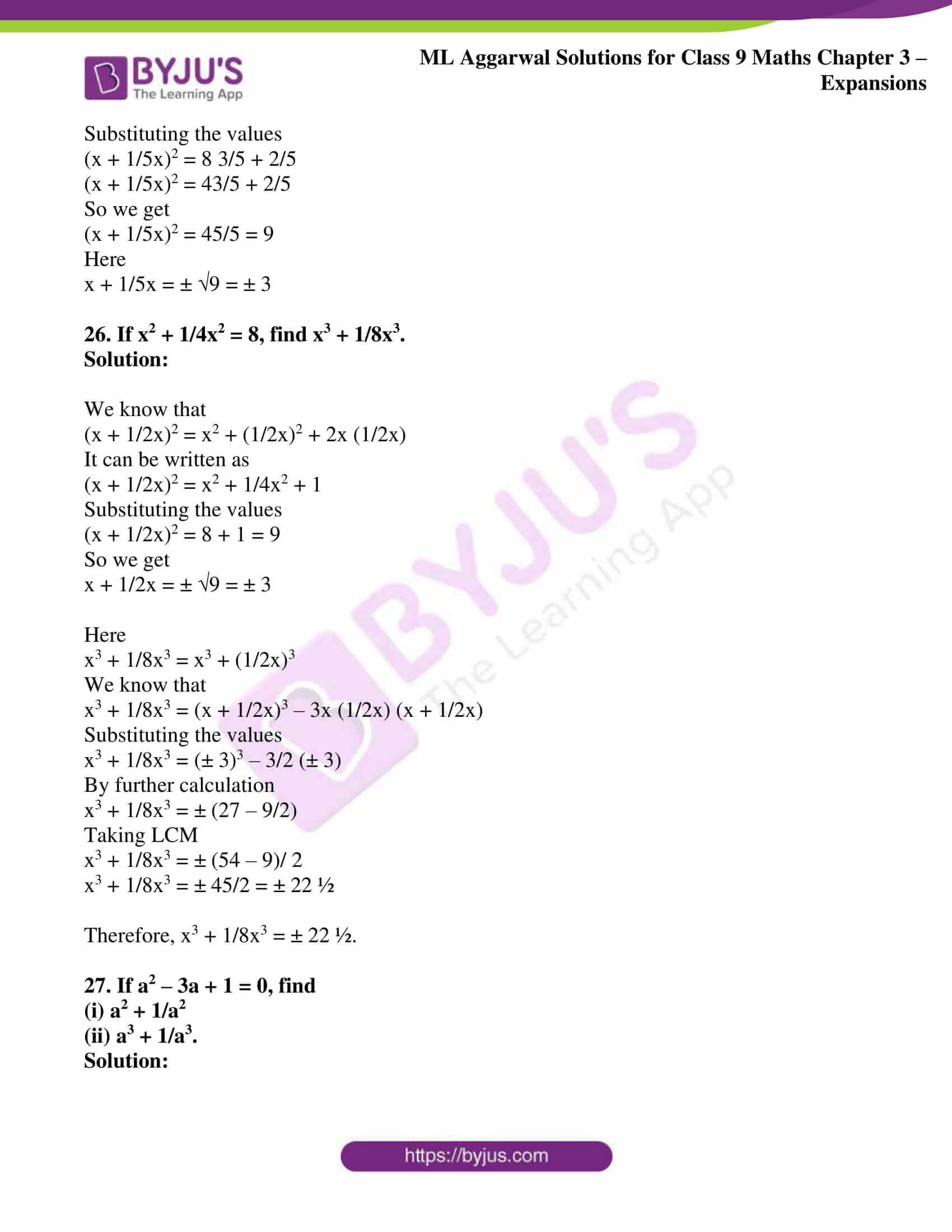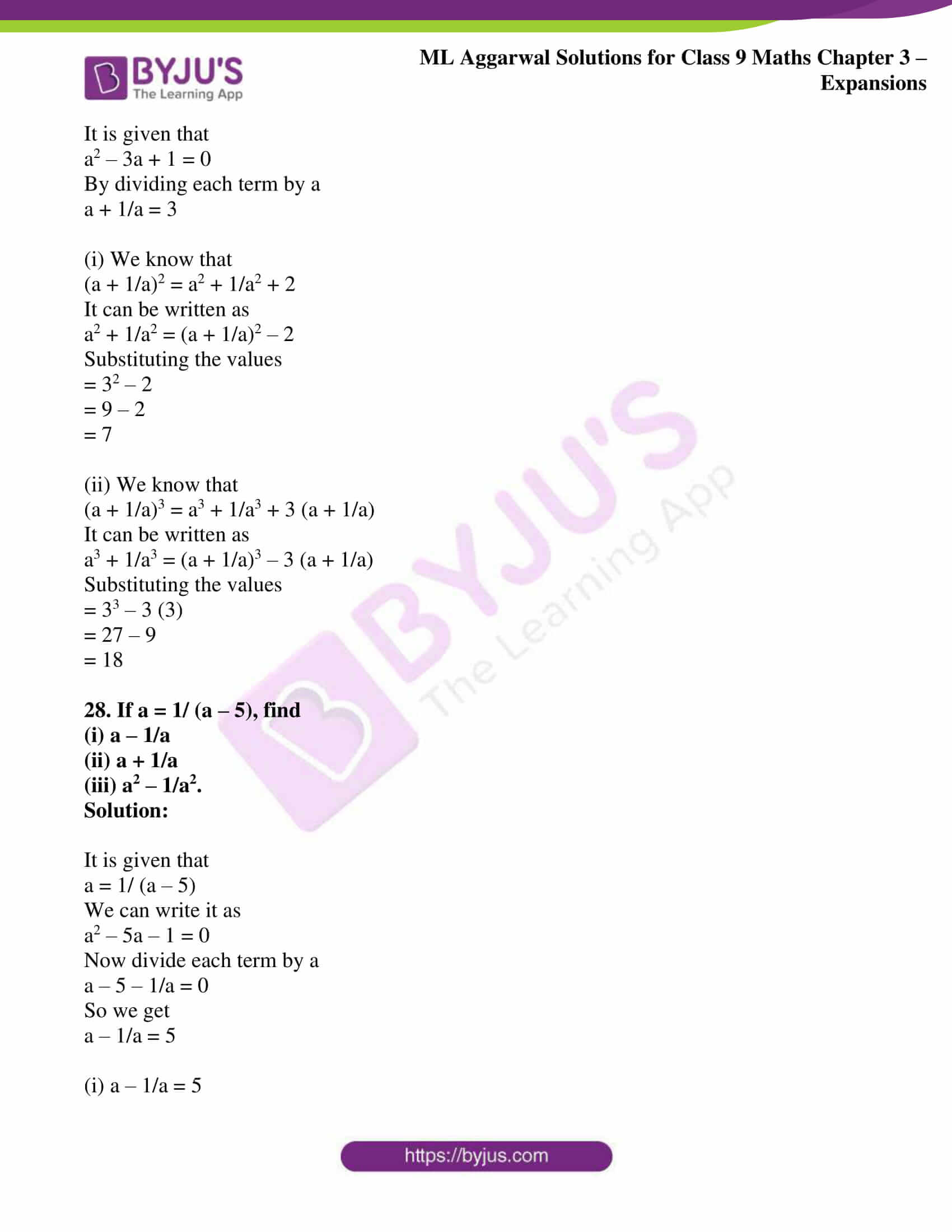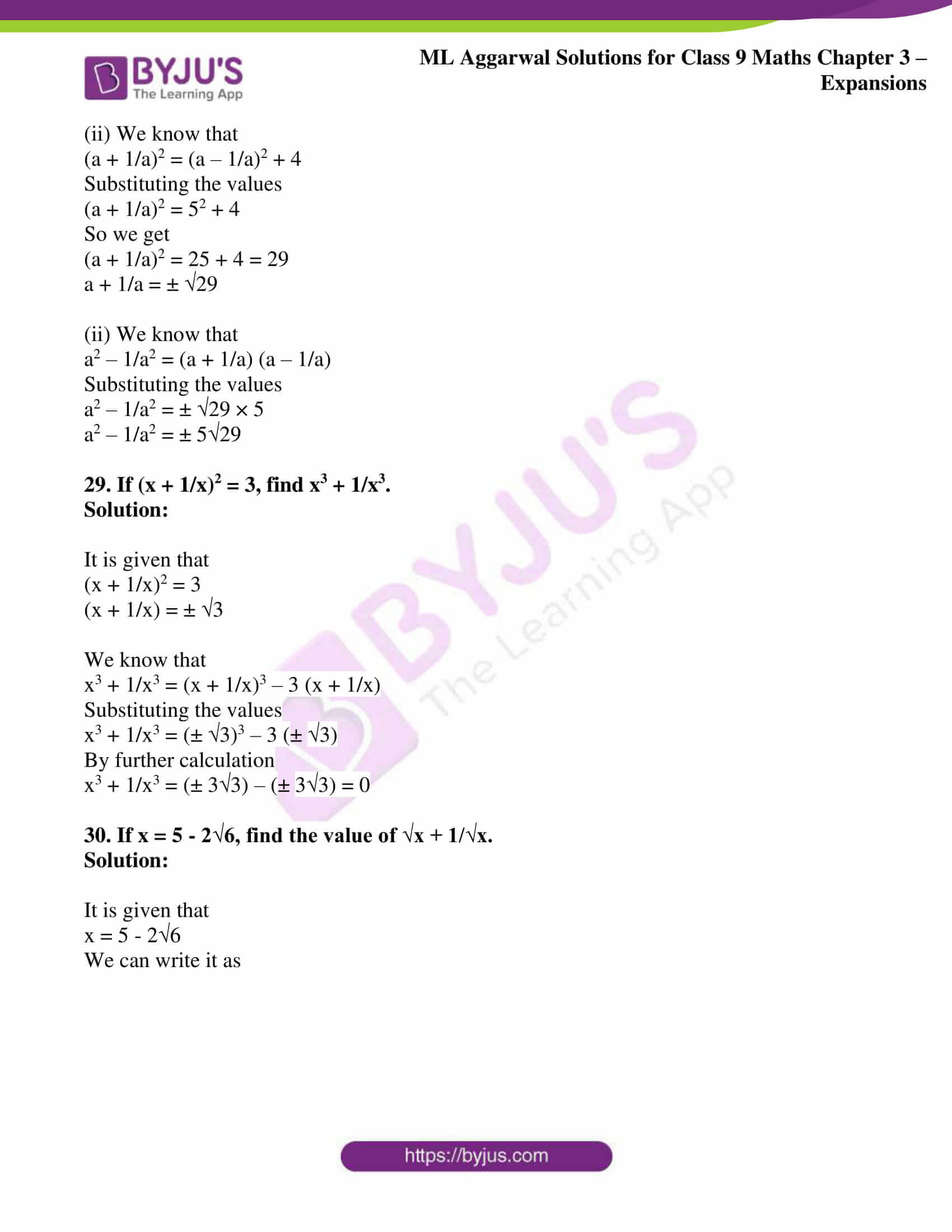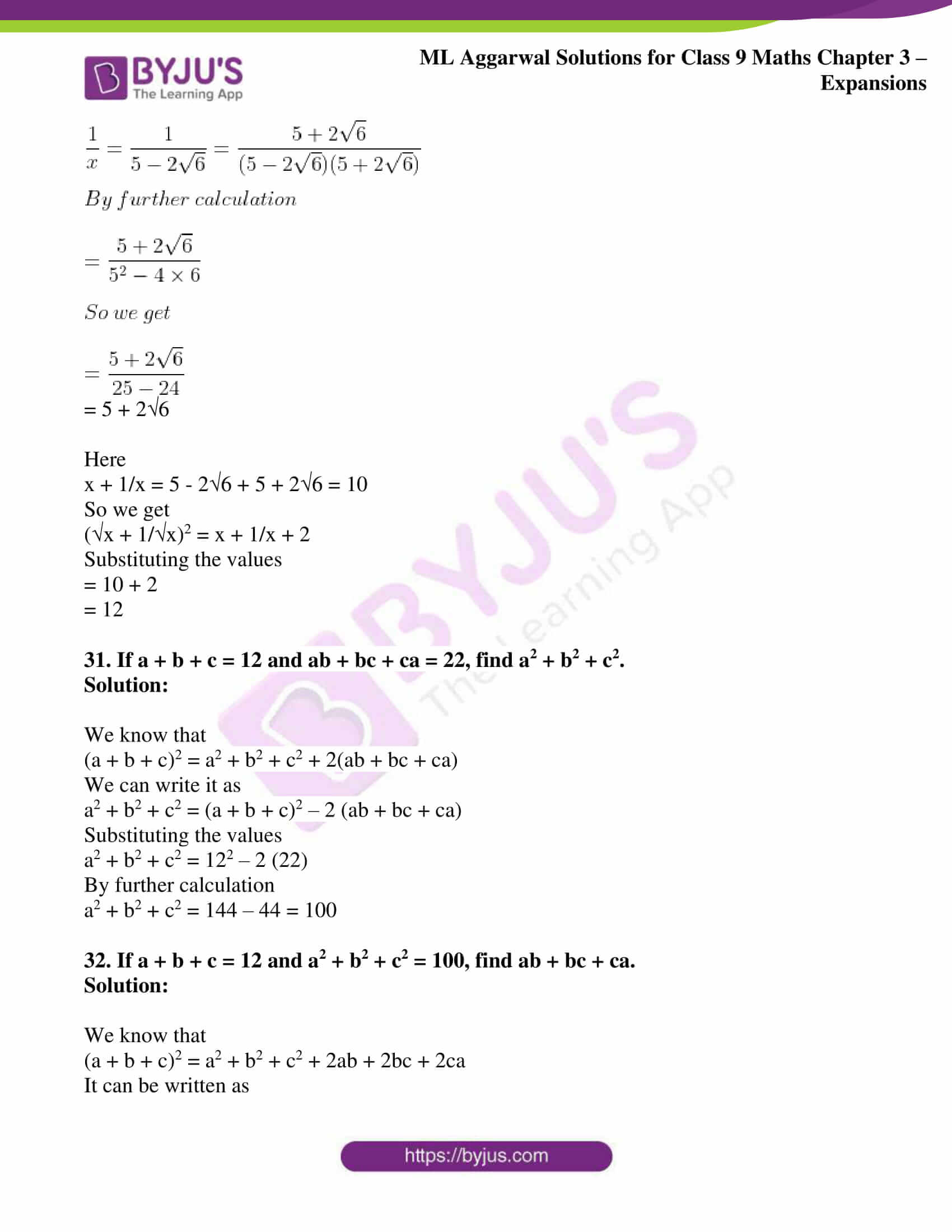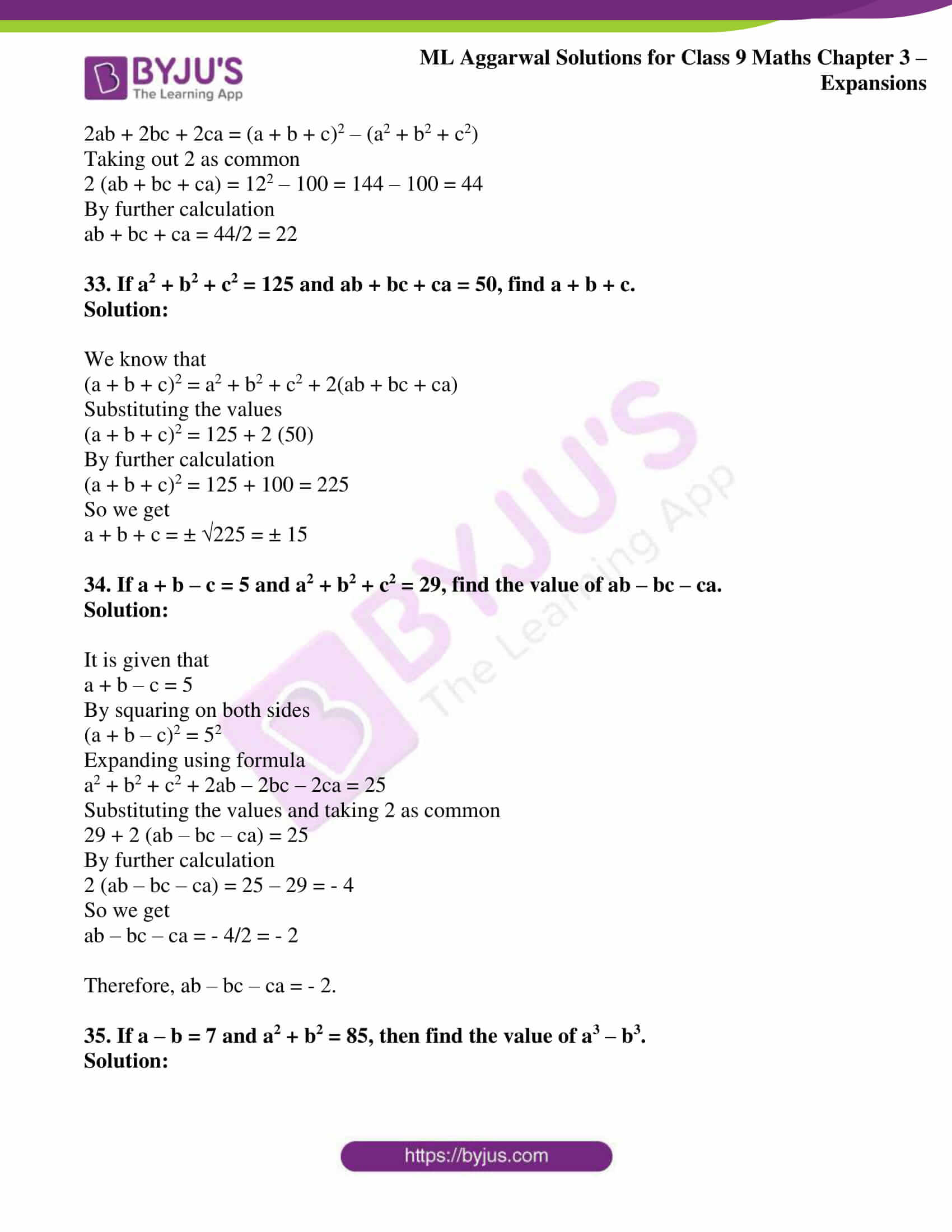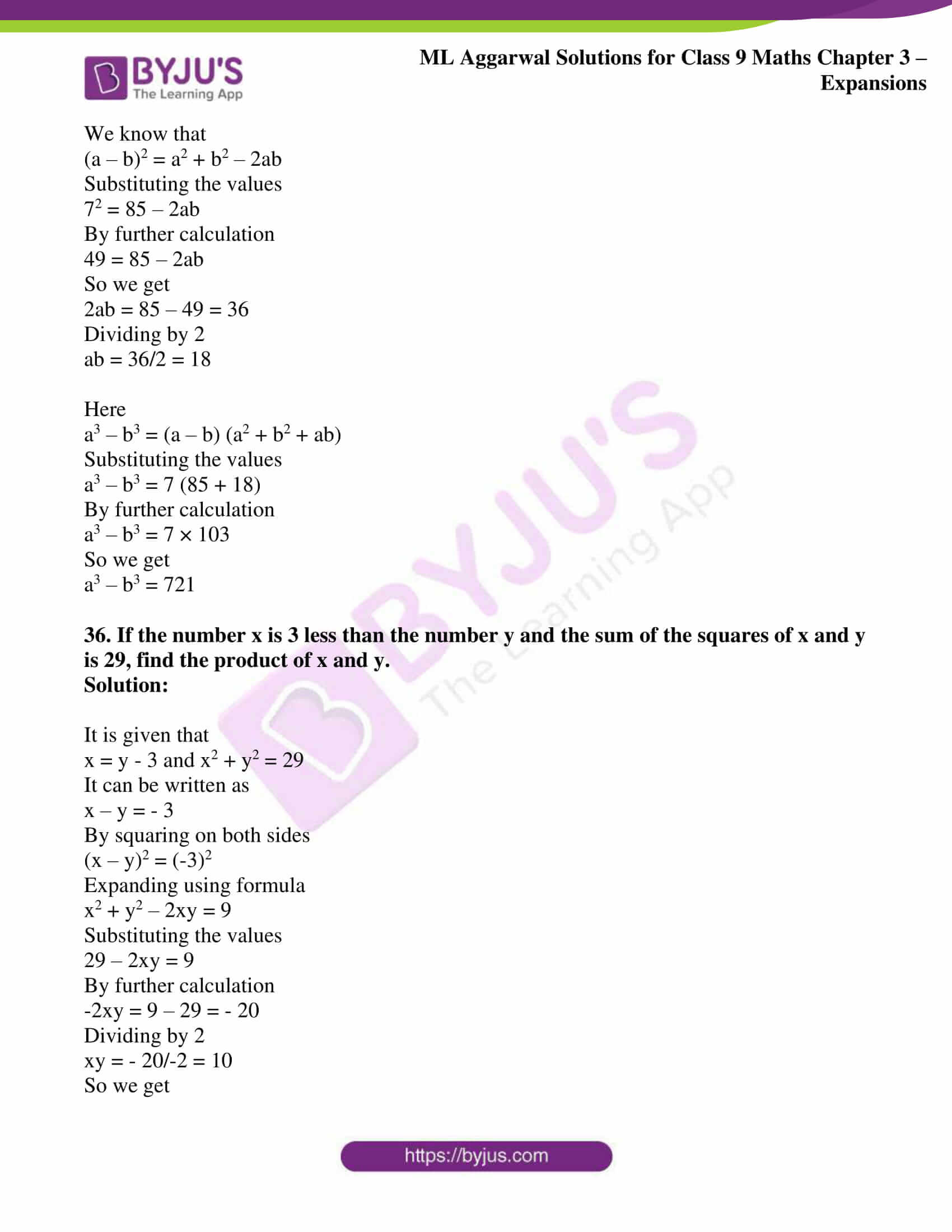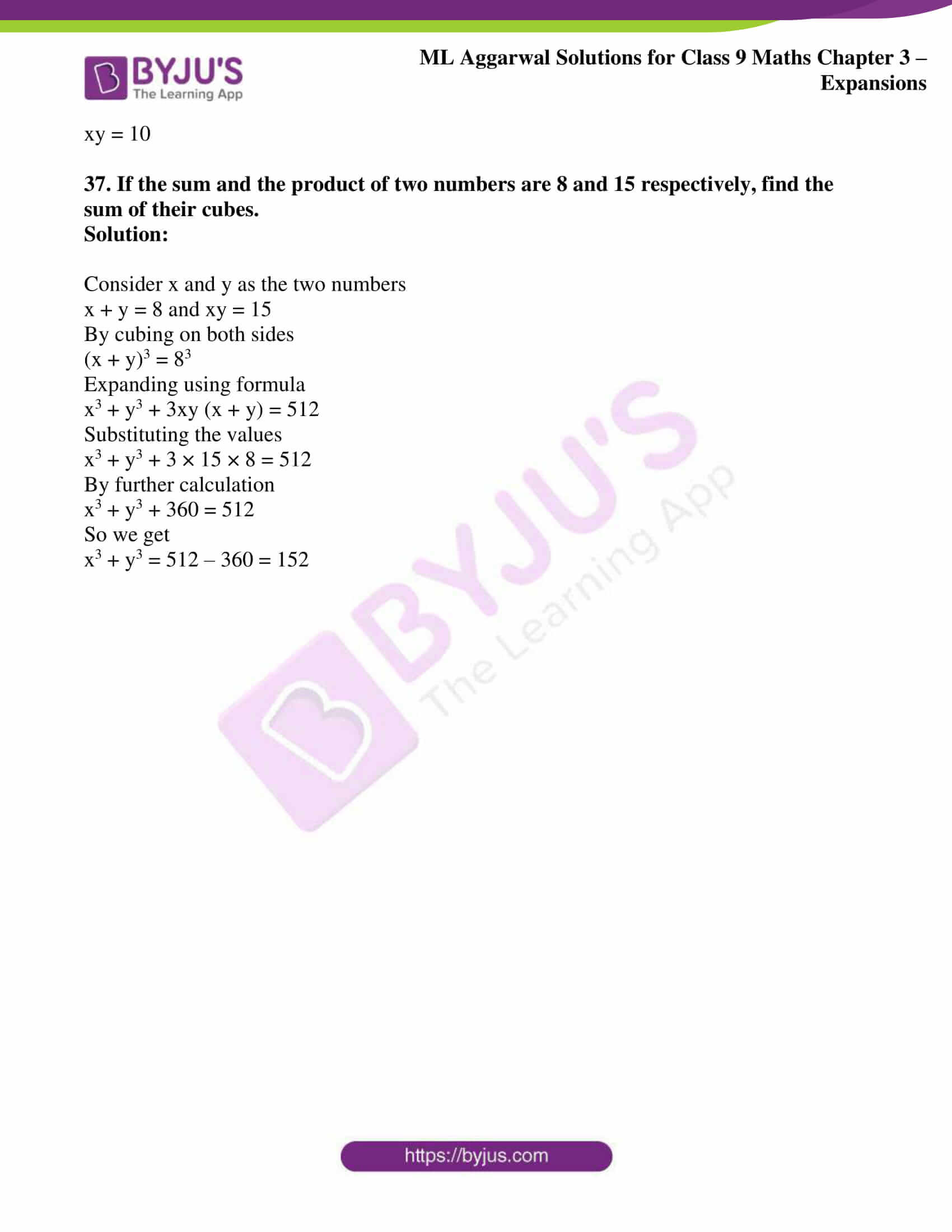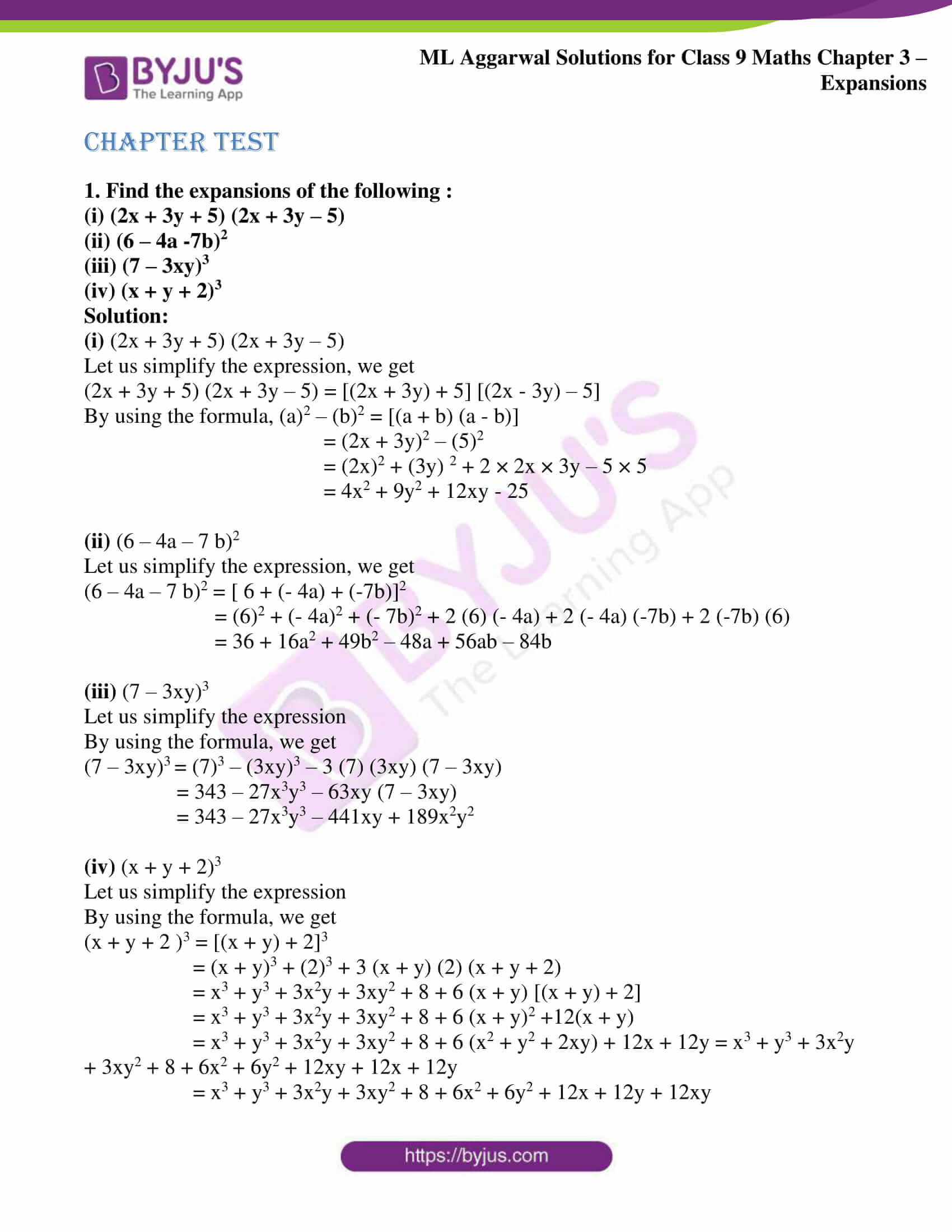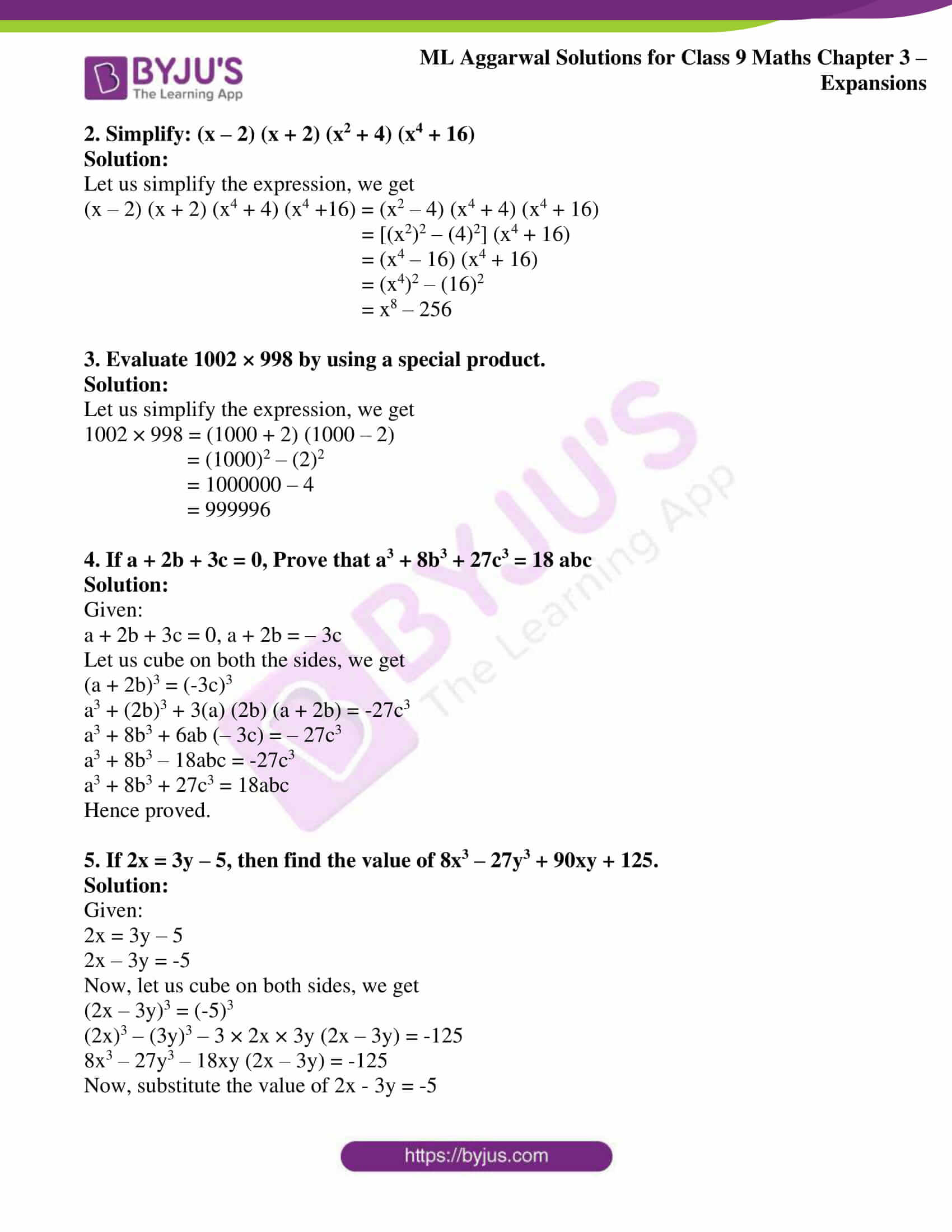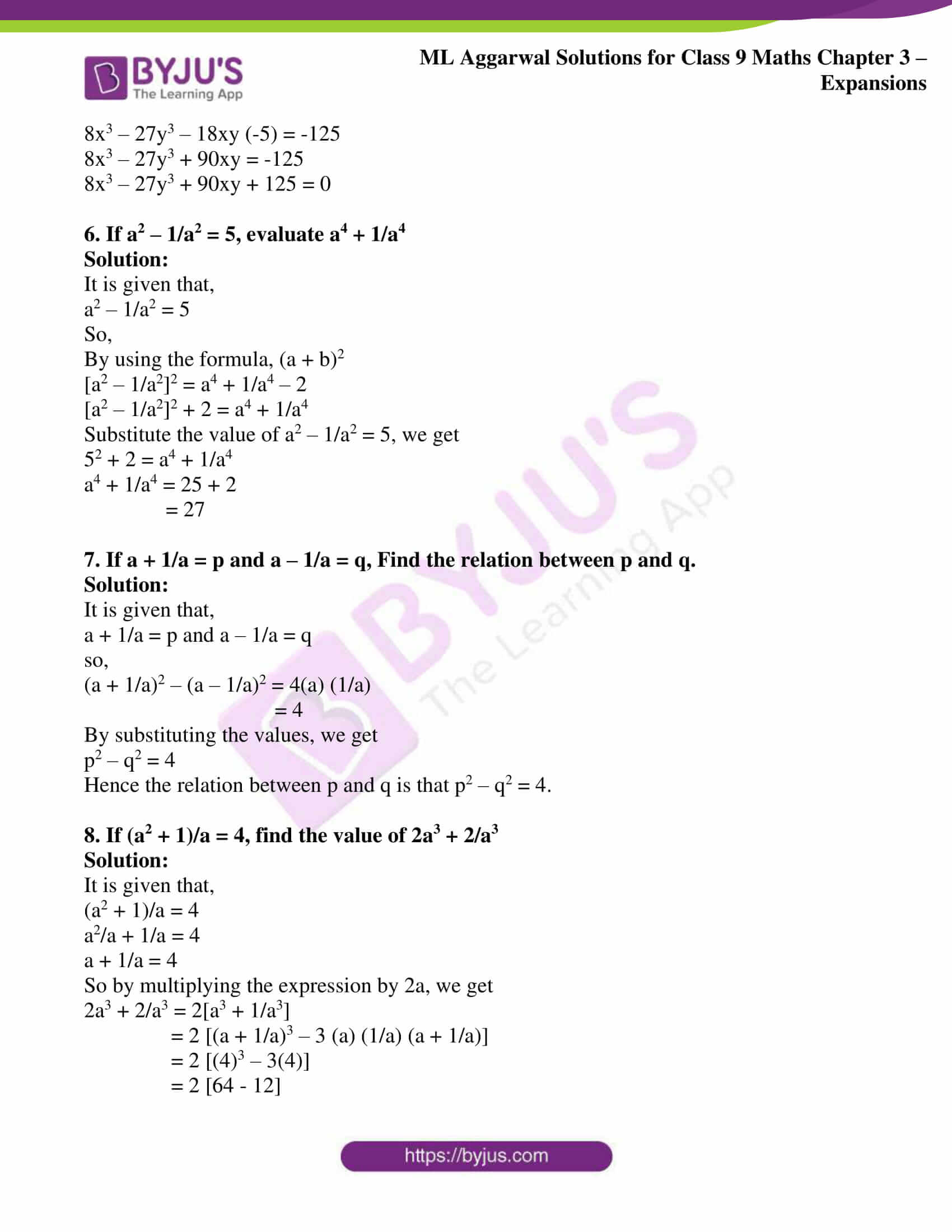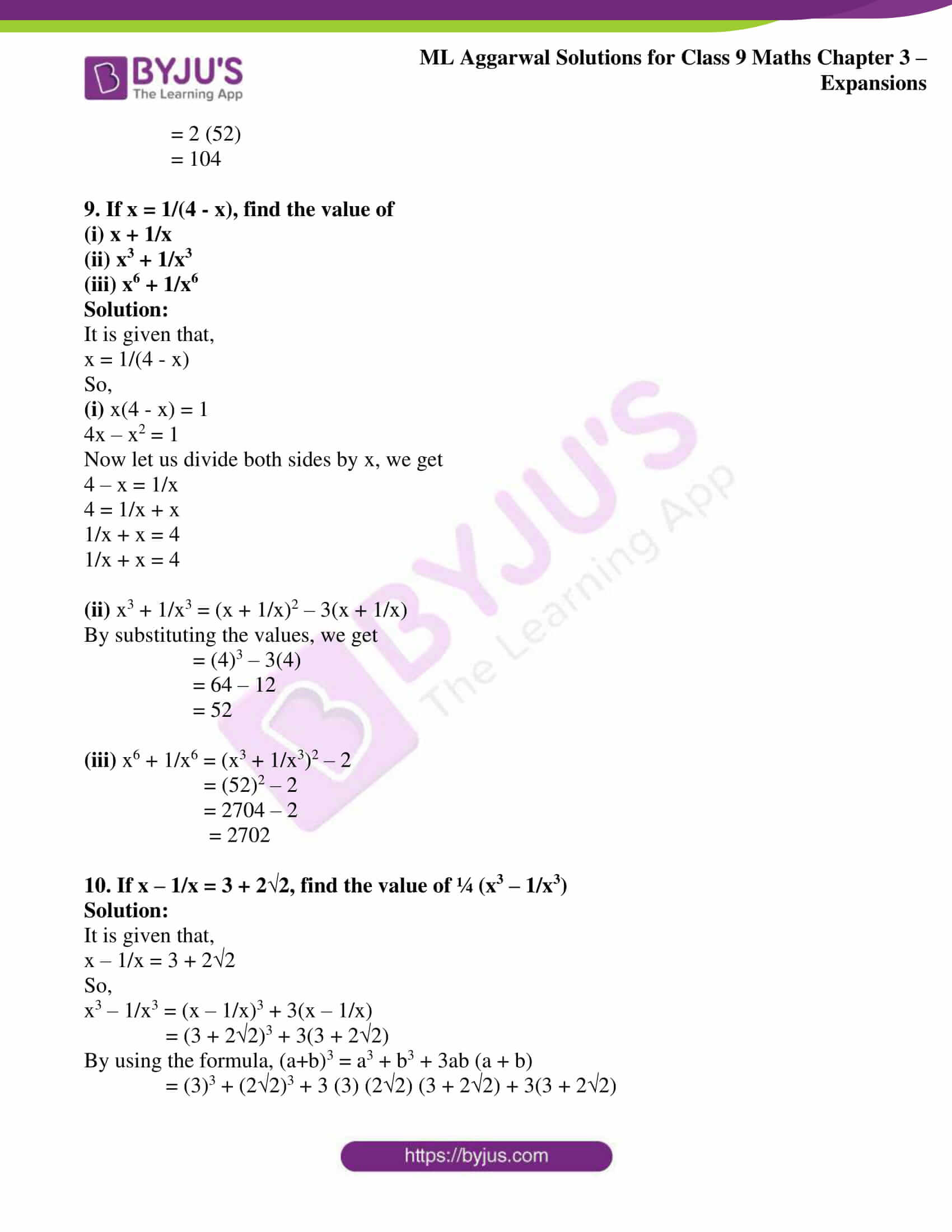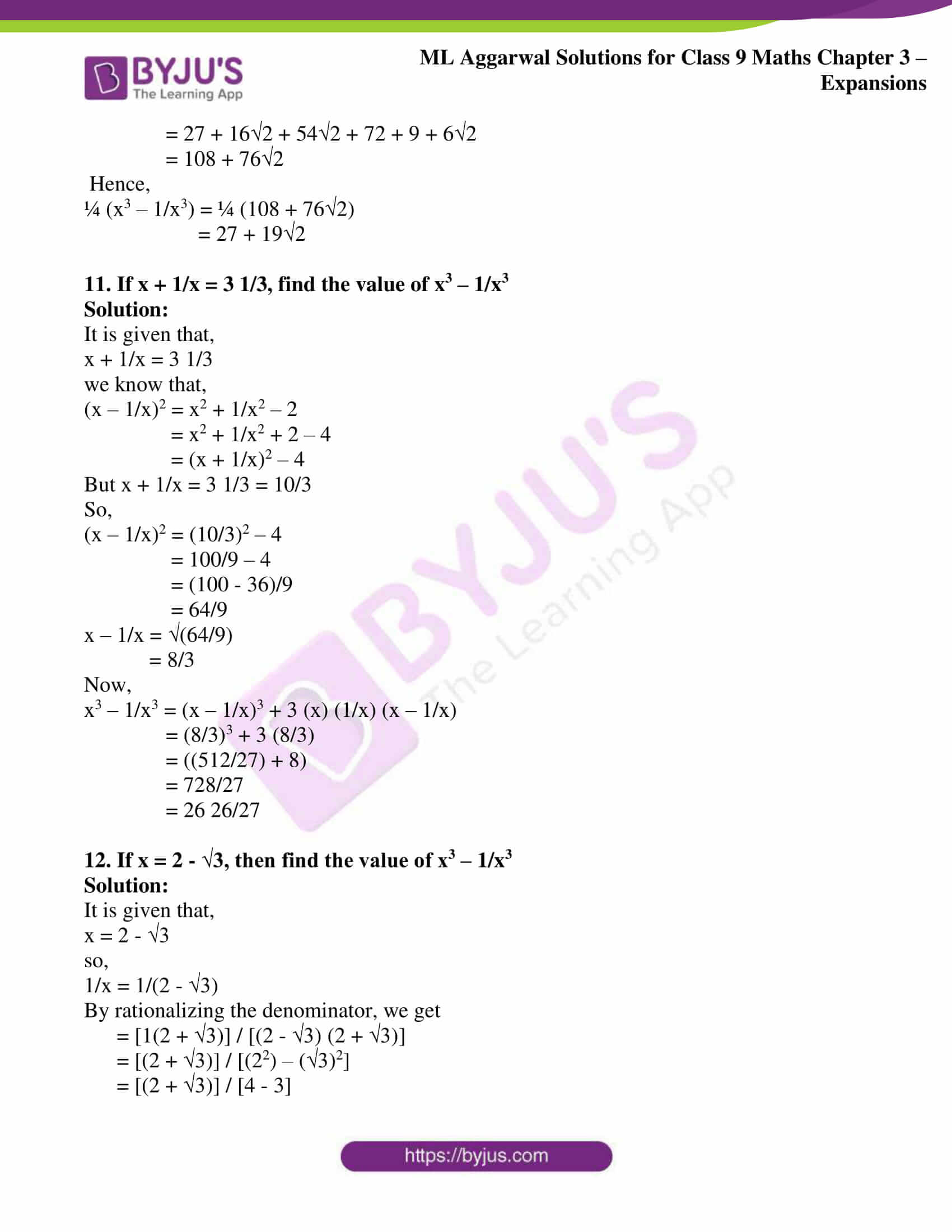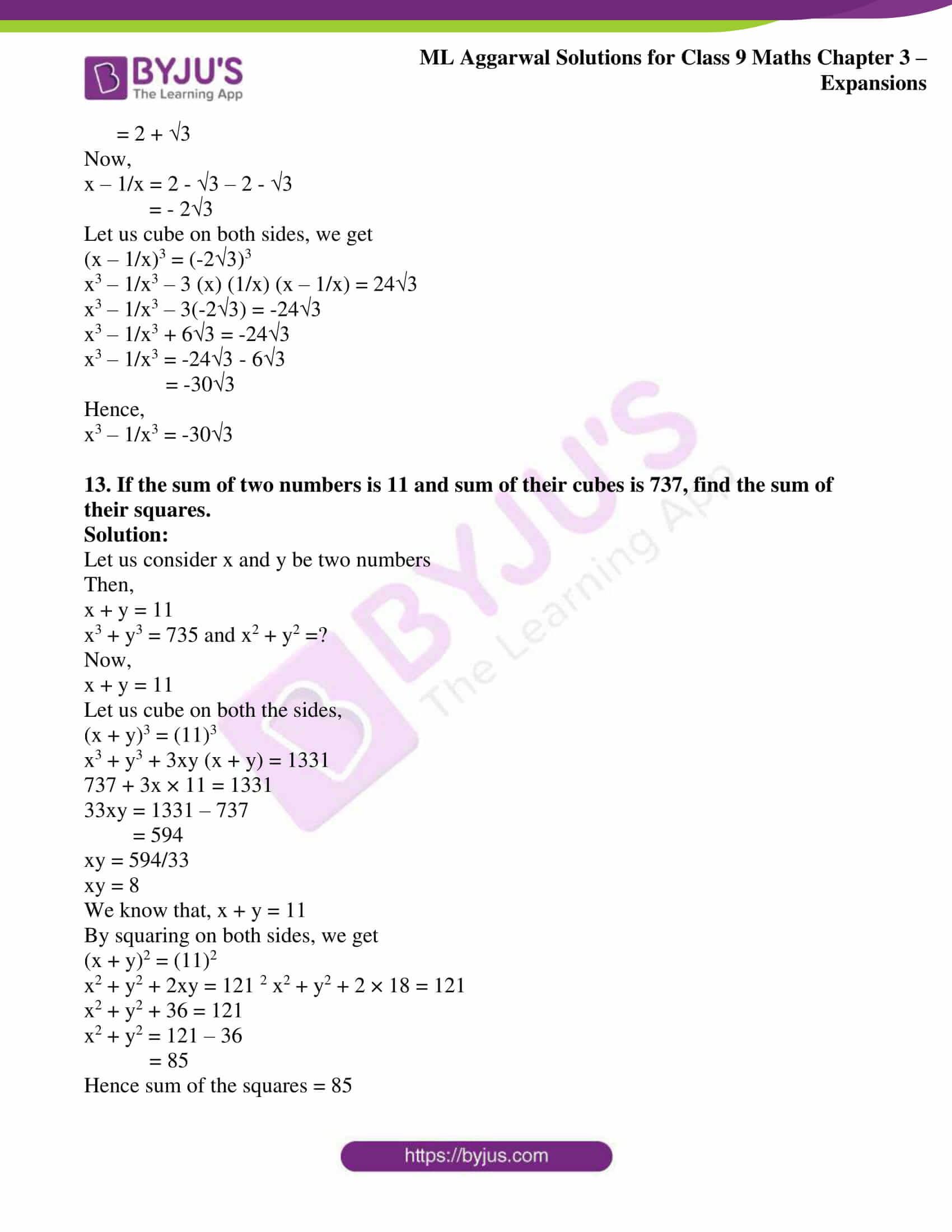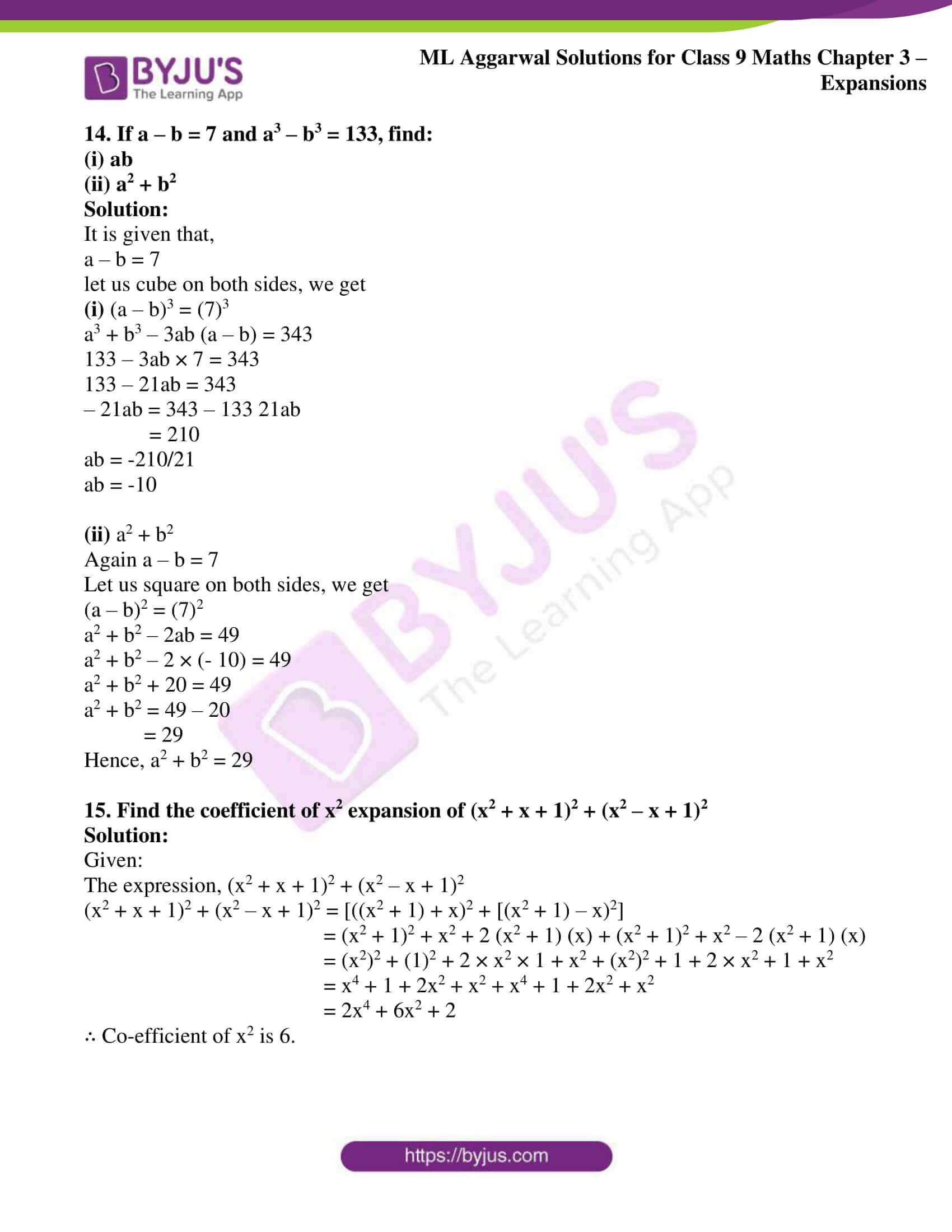### Access answers to ML Aggarwal Solutions for Class 9 Maths Chapter 3 – Expansions

Exercise 3.1

By using standard formulae, expand the following (1 to 9):

1. (i) (2x + 7y)2

(ii) (1/2 x + 2/3 y)2

Solution:

(i) (2x + 7y)2

It can be written as

= (2x)2 + 2 × 2x × 7y + (7y)2

So we get

= 4x2 + 28xy + 49y2

(ii) (1/2 x + 2/3 y)2

It can be written as

= (1/2 x)2 + 2 × ½x + 2/3y + (2/3 y)2

So we get

= ¼ x2 + 2/3 xy + 4/9 y2

2. (i) (3x + 1/2x)2

(ii) (3x2y + 5z)2

Solution:

(i) (3x + 1/2x)2

It can be written as

= (3x)2 + 2 × 3x × 1/2x + (1/2x)2

So we get

= 9x2 + 3 + 1/4x2

= 9x2 + 1/4x2 + 3

(ii) (3x2y + 5z)2

It can be written as

= (3x2y)2 + 2 × 3x2y × 5z + (5z)2

So we get

= 9x4y2 + 30x2yz + 25z2

3. (i) (3x – 1/2x)2

(ii) (1/2 x – 3/2 y)2

Solution:

(i) (3x – 1/2x)2

It can be written as

= (3x)2 – 2 × 3x × 1/2x + (1/2x)2

So we get

= 9x2 – 3 + 1/4x2

= 9x2 + 1/4x2 – 3

(ii) (1/2 x – 3/2 y)2

It can be written as

= (1/2 x)2 + (3/2 y)2 – 2 × ½ x × 3/2 y

So we get

= ¼ x2 + 9/4 y2 – 3/2 xy

= ¼ x2 – 3/2 xy + 9/4 y2

4. (i) (x + 3) (x + 5)

(ii) (x + 3) (x – 5)

(iii) (x – 7) (x + 9)

(iv) (x – 2y) (x – 3y)

Solution:

(i) (x + 3) (x + 5)

By further calculation

= x2 + (3 + 5) x + 3 × 5

So we get

= x2 + 8x + 15

(ii) (x + 3) (x – 5)

By further calculation

= x2 + (3 – 5)x – 3 × 5

So we get

= x2 – 2x – 15

(iii) (x – 7) (x + 9)

By further calculation

= x2 – (7 – 9)x – 7 × 9

So we get

= x2 + 2x – 63

(iv) (x – 2y) (x – 3y)

By further calculation

= x2 – (2y + 3y)x + 2y × 3y

So we get

= x2 – 5xy + 6y2

5. (i) (x – 2y – z)2

(ii) (2x – 3y + 4z)2

Solution:

(i) (x – 2y – z)2

It can be written as

= [x + (-2y) + (-z)]2

By further calculation

= (x)2 + (-2y)2 + (-z)2 + 2 × x × (-2y) + 2 × (-2y) × (-z) + 2 × (-z) × x

So we get

= x2 + 4y2 + z2 – 4xy + 4yz – 2zx

(ii) (2x – 3y + 4z)2

It can be written as

= [2x + (-3y) + 4z]2

By further calculation

= (2x)2 + (-3y)2 + (4z)2 + 2 × 2x × (-3y) + 2 × (-3y) × 4z + 2 × 4z × 2x

So we get

= 4x2 + 9y2 + 16z2 -12xy – 24yz + 16zx

6. (i) (2x + 3/x – 1)2

(ii) (2/3 x – 3/2x – 1)2

Solution:

(i) (2x + 3/x – 1)2

It can be written as

= [2x + 3/x + (-1)]2

By further calculation

= (2x)2 + (3/x)2 + (-1)2 + 2 ×2x × 3/x + 2 × 3/x × (-1) + 2 × (-1) × 2x

So we get

= 4x2 + 9/x2 + 1 + 12 – 6/x – 4x

= 4x2 + 9/x2 + 13 – 6/x – 4x

(ii) (2/3 x – 3/2x – 1)2

It can be written as

= [2/3 x – 3/2x – 1]2

By further calculation

= (2/3 x)2 + (-3/2x)2 + (-1)2 + 2 × 2/3 x × (-3/2x) + 2 × (-3/2x) × (-1) + 2 × (-1) × (2/3 x)

So we get

= 4/9 x2 + 9/4x2 + 1 – 2 + 3/x – 4/3 x

= 4/9 x2 + 9/4x2 – 1 – 4/3 x + 3/x

7. (i) (x + 2)3

(ii) (2a + b)3

Solution:

(i) (x + 2)3

It can be written as

= x3 + 23 + 3 × x × 2 (x + 2)

By further calculation

= x3 + 8 + 6x (x + 2)

So we get

= x3 + 8 + 6x2 + 12x

= x3 + 6x2 + 12x + 8

(ii) (2a + b)3

It can be written as

= (2a)3 + b3 + 3 × 2a × b (2a + b)

By further calculation

= 8a3 + b3 + 6ab (2a + b)

So we get

= 8a3 + b3 + 12a2b + 6ab2

8. (i) (3x + 1/x)3

(ii) (2x – 1)3

Solution:

(i) (3x + 1/x)3

It can be written as

= (3x)3 + (1/x)3 + 3 × 3x × 1/x (3x + 1/x)

By further calculation

= 27x3 + 1/x3 + 9 (3x + 1/x)

So we get

= 27x3 + 1/x3 + 27x + 9/x

(ii) (2x – 1)3

It can be written as

= (2x)3 – 13 – 3 × 2x × 1 (2x – 1)

By further calculation

= 8x3 – 1 – 6x (2x – 1)

So we get

= 8x3 – 1 – 12x2 + 6x

= 8x3 – 12x2 + 6x – 1

9. (i) (5x – 3y)3

(ii) (2x – 1/3y)3

Solution:

(i) (5x – 3y)3

It can be written as

= (5x)3 – (3y)3 – 3 × 5x × 3y (5x – 3y)

By further calculation

= 125x3 – 27y3 – 45xy (5x – 3y)

So we get

= 125x3 – 27y3 – 225x2y + 135xy2

(ii) (2x – 1/3y)3

It can be written as

= (2x)3 – (1/3y)3 – 3 × 2x × 1/3y (2x – 1/3y)

By further calculation

= 8x3 – 1/27y3 – 2x/y (2x – 1/3y)

So we get

= 8x3 – 1/27y3 – 4x2/y + 2x/3y2

Simplify the following (10 to 19):

10. (i) (a + b)2 + (a – b)2

(ii) (a + b)2 – (a – b)2

Solution:

(i) (a + b)2 + (a – b)2

It can be written as

= (a2 + b2 + 2ab) + (a2 +b2 – 2ab)

By further calculation

= a2 + b2 + 2ab + a2 + b2 – 2ab

So we get

= 2a2 + 2b2

Taking 2 as common

= 2 (a2 + b2)

(ii) (a + b)2 – (a – b)2

It can be written as

= (a2 + b2 + 2ab) – (a2 + b2 – 2ab)

By further calculation

= a2 + b2 + 2ab – a2 – b2 + 2ab

So we get

= 4ab

11. (i) (a + 1/a)2 + (a – 1/a)2

(ii) (a + 1/a)2 – (a – 1/a)2

Solution:

(i) (a + 1/a)2 + (a – 1/a)2

It can be written as

= [a2 + (1/a)2 + 2 × a × 1/a] + [a2 + (1/a)2 – 2 × a × 1/a]

By further calculation

= [a2 + 1/a2 + 2] + [a2 + 1/a2 – 2]

So we get

= a2 + 1/a2 + 2 + a2 + 1/a2 – 2

= 2a2 + 2/a2

Taking 2 as common

= 2 (a2 + 1/a2)

(ii) (a + 1/a)2 – (a – 1/a)2

It can be written as

= [a2 + (1/a)2 + 2 × a × 1/a] – [a2 + (1/a)2 – 2 × a × 1/a]

By further calculation

= [a2 + 1/a2 + 2] – [a2 + 1/a2 – 2]

So we get

= a2 + 1/a2 + 2 – a2 – 1/a2 + 2

= 4

12. (i) (3x – 1)2 – (3x – 2) (3x + 1)

(ii) (4x + 3y)2 – (4x – 3y)2 – 48xy

Solution:

(i) (3x – 1)2 – (3x – 2) (3x + 1)

It can be written as

= [(3x)2 + 12 – 2 × 3x × 1] – [(3x)2 – (2 – 1) (3x) – 2 × 1]

By further calculation

= [9x2 + 1 – 6x] – [9x2 – 3x – 2]

So we get

= 9x2 + 1 – 6x – 9x2 + 3x + 2

= -3x + 3

= 3 – 3x

(ii) (4x + 3y)2 – (4x – 3y)2 – 48xy

It can be written as

= [(4x)2 + (3y)2 + 2 × 4x × 4y] – [(4x)2 + (3y)2 – 2 × 4x × 3y] – 48xy

By further calculation

= [16x2 + 9y2 + 24xy] – [16x2 + 9y2 – 24xy] – 48xy

So we get

= 16x2 + 9y2 + 24xy – 16x2 – 9y2 + 24xy – 48xy

= 0

13. (i) (7p + 9q) (7p – 9q)

(ii) (2x – 3/x) (2x + 3/x)

Solution:

(i) (7p + 9q) (7p – 9q)

It can be written as

= (7p)2 – (9q)2

= 49p2 – 81q2

(ii) (2x – 3/x) (2x + 3/x)

It can be written as

= (2x)2 – (3/x)2

= 4x2 – 9/x2

14. (i) (2x – y + 3) (2x – y – 3)

(ii) (3x + y – 5) (3x – y – 5)

Solution:

(i) (2x – y + 3) (2x – y – 3)

It can be written as

= [(2x – y) + 3] [(2x – y) – 3]

= (2x – y)2 – 32

By further calculation

= (2x)2 +y2 – 2 × 2x × y – 9

So we get

= 4x2 + y2 – 4xy – 9

(ii) (3x + y – 5) (3x – y – 5)

It can be written as

= [(3x – 5) + y] [(3x – 5) – y]

= (3x – 52) – y2

By further calculation

= (3x)2 + 52 – 2 × 3x × 5 – y2

So we get

= 9x2 + 25 – 30x – y2

= 9x2 – y2 – 30x + 25

15. (i) (x + 2/x – 3) (x – 2/x – 3)

(ii) (5 – 2x) (5 + 2x) (25 + 4x2)

Solution:

(i) (x + 2/x – 3) (x – 2/x – 3)

It can be written as

= [(x – 3) + (2/x)] [(x – 3) – (2/x)]

= (x – 3)2 – (2/x)2

Expanding using formula

= x2 + 9 – 2 × x × 3 – 4/x2

By further calculation

= x2 + 9 – 6x – 4/x2

So we get

= x2 – 4/x2 – 6x + 9

(ii) (5 – 2x) (5 + 2x) (25 + 4x2)

It can be written as

= [52 – (2x)2] (25 + 4x2)

By further calculation

= (25 – 4x2) (25 + 4x2)

So we get

= 252 – (4x2)2

= 625 – 16x4

16. (i) (x + 2y + 3) (x + 2y + 7)

(ii) (2x + y + 5) (2x + y – 9)

(iii) (x – 2y – 5) (x – 2y + 3)

(iv) (3x – 4y – 2) (3x – 4y – 6)

Solution:

(i) (x + 2y + 3) (x + 2y + 7)

Consider x + 2y = a

(a + 3) (a + 7) = a2 + (3 + 7) a + 3 × 7

By further calculation

= a2 + 10a + 21

Substituting the value of a

= (x + 2y)2 + 10 (x + 2y) + 21

By expanding using formula

= x2 + 4y2 + 2 × x × 2y + 10x + 20y + 21

So we get

= x2 + 4y2 + 4xy + 10x + 20y + 21

(ii) (2x + y + 5) (2x + y – 9)

Consider 2x + y = a

(a + 5) (a – 9) = a2 + (5 – 9) a + 5 × (-9)

By further calculation

= a2 – 4a – 45

Substituting the value of a

= (2x + y)2 – 4 (2x + y) – 45

By expanding using formula

= 4x2 + y2 + 2 × 2x × y – 8x – 4y – 45

So we get

= 4x2 + y2 + 4xy – 8x – 4y – 45

(iii) (x – 2y – 5) (x – 2y + 3)

Consider x – 2y = a

(a – 5) (a + 3) = a2 + (- 5 + 3) a + (-5) (3)

By further calculation

= a2 – 2a – 15

Substituting the value of a

= (x – 2y)2 – 2 (x – 2y) – 15

By expanding using formula

= x2 + 4y2 – 2 × x × 2y – 2x + 4y – 15

So we get

= x2 + 4y2 – 4xy – 2x + 4y – 15

(iv) (3x – 4y – 2) (3x – 4y – 6)

Consider 3x – 4y = a

(a – 2) (a – 6) = a2 (- 2 – 6)a + (-2) (-6)

By further calculation

= a2 – 8a + 12

Substituting the value of a

= (3x – 4y)2 – 8 (3x – 4y) + 12

Expanding using formula

= 9x2 + 16y2 – 2 × 3x × 4y – 24x + 32y + 12

So we get

= 9x2 + 16y2 – 24xy – 24x + 32y + 12

17. (i) (2p + 3q) (4p2 – 6pq + 9q2)

(ii) (x + 1/x) (x2 – 1 + 1/x2)

Solution:

(i) (2p + 3q) (4p2 – 6pq + 9q2)

It can be written as

= (2p + 3q) [(2p)2 – 2p × 3q + (3q)2]

By further simplification

= (2p)3 + (3q)3

= 8p3 + 27q3

(ii) (x + 1/x) (x2 – 1 + 1/x2)

It can be written as

= (x + 1/x) [x2 – x × 1/x + (1/x)2]

By further simplification

= x3 + (1/x)3

= x3 + 1/x3

18. (i) (3p – 4q) (9p2 + 12pq + 16q2)

(ii) (x – 3/x) (x2 + 3 + 9/x2)

Solution:

(i) (3p – 4q) (9p2 + 12pq + 16q2)

It can be written as

= (3p – 4q) [(3p)2 + 3p × 4q + (4q)2]

By further simplification

= (3p)3 – (4q)3

= 27p3 – 64q3

(ii) (x – 3/x) (x2 + 3 + 9/x2)

It can be written as

= (x – 3/x) [x2 + x × 3/x + (3/x)2]

By further simplification

= x3 – (3/x)3

= x3 – 27/x3

19. (2x + 3y + 4z) (4x2 + 9y2 + 16z2 – 6xy – 12yz – 8zx).

Solution:

(2x + 3y + 4z) (4x2 + 9y2 + 16z2 – 6xy – 12yz – 8zx)

It can be written as

= (2x + 3y + 4z) ((2x)2 + (3y)2 + (4z)2 – 2x × 3y – 3y × 4z – 4z × 2x)

By further calculation

= (2x)3 + (3y)3 + (4z)3 – 3 × 2x × 3y × 4z

So we get

= 8x3 + 27y3 + 64z3 – 72xyz

20. Find the product of the following:

(i) (x + 1) (x + 2) (x + 3)

(ii) (x – 2) (x – 3) (x + 4)

Solution:

(i) (x + 1) (x + 2) (x + 3)

It can be written as

= x3 + (1 + 2 + 3)x2 + (1 × 2 + 2 × 3 + 3 × 1) x + 1 × 2 × 3

By further calculation

= x3 + 6x2 + (2 + 6 + 3)x + 6

So we get

= x3 + 6x2 + 11x + 6

(ii) (x – 2) (x – 3) (x + 4)

It can be written as

= x3 + (- 2 – 3 + 4) x2 + [(-2) × (-3) + (-3) × 4 + 4 × (-2)]x + (-2) (-3) (4)

By further calculation

= x3 – x2 + (6 – 12 – 8)x + 24

= x3 – x2 – 14x + 24

21. Find the coefficient of x2 and x in the product of (x – 3) (x + 7) (x – 4).

Solution:

It is given that

(x – 3) (x + 7) (x – 4)

By further calculation

= x3 + (- 3 + 7 – 4) x2 + [(-3) (7) + 7 × (-4) + (-4) (-3) + (-3) (7) (-4)]

It can be written as

= x3 + 0x2 + (- 21 – 28 + 12) x + 84

So we get

= x3 + 0x2 – 37x + 84

Hence, coefficient of x2 is zero and coefficient of x is – 3.

22. If a2 + 4a + x = (a + 2)2, find the value of x.

Solution:

It is given that

a2 + 4a + x = (a + 2)2

By expanding using formula

a2 + 4a + x = a2 + 22 + 2 × a × 2

By further calculation

a2 + 4a + x = a2 + 4 + 4a

So we get

x = a2 + 4 + 4a – a2 – 4a

x = 4

23. Use (a + b)2 = a2 + 2ab + b2 to evaluate the following:

(i) (101)2

(ii) (1003)2

(iii) (10.2)2

Solution:

(i) (101)2

It can be written as

= (100 + 1)2

Expanding using formula

= 1002 + 12 + 2 × 100 × 1

By further calculation

= 10000 + 1 + 200

= 10201

(ii) (1003)2

It can be written as

= (1000 + 3)2

Expanding using formula

= 10002 + 32 + 2 × 1000 × 3

By further calculation

= 1000000 + 9 + 6000

= 1006009

(iii) (10.2)2

It can be written as

= (10 + 0.2)2

Expanding using formula

= 102 + 0.22 + 2 × 10 × 0.2

By further calculation

= 100 + 0.04 + 4

= 104.04

24. Use (a – b)2 = a2 – 2ab – b2 to evaluate the following:

(i) (99)2

(ii) (997)2

(iii) (9.8)2

Solution:

(i) (99)2

It can be written as

= (100 – 1)2

Expanding using formula

= 1002 – 2 × 100 × 1 + 12

By further calculation

= 10000 – 200 + 1

= 9801

(ii) (997)2

It can be written as

= (1000 – 3)2

Expanding using formula

= 10002 – 2 × 1000 × 3 + 32

By further calculation

= 1000000 – 6000 + 9

= 994009

(iii) (9.8)2

It can be written as

= (10 – 0.2)2

Expanding using formula

= 102 – 2 × 10 × 0.2 + 0.22

By further calculation

= 100 – 4 + 0.04

= 96.04

25. By using suitable identities, evaluate the following:

(i) (103)3

(ii) (99)3

(iii) (10.1)3

Solution:

(i) (103)3

It can be written as

= (100 + 3)3

Expanding using formula

= 1003 + 33 + 3 × 100 × 3 (100 + 3)

By further calculation

= 1000000 + 27 + 900 × 103

So we get

= 1000000 + 27 + 92700

= 1092727

(ii) (99)3

It can be written as

= (100 – 1)3

Expanding using formula

= 1003 – 13 – 3 × 100 × 1 (100 – 1)

By further calculation

= 1000000 – 1 – 300 × 99

So we get

= 1000000 – 1 – 29700

= 1000000 – 29701

= 970299

(iii) (10.1)3

It can be written as

= (10 + 0.1)3

Expanding using formula

= 103 + 0.13 + 3 × 10 × 0.1 (10 + 0.1)

By further calculation

= 1000 + 0.001 + 3 × 10.1

So we get

= 1000 + 0.001 + 30.3

= 1030.301

26. If 2a – b + c = 0, prove that 4a2 – b2 + c2 + 4ac = 0.

Solution:

It is given that

2a – b + c = 0

2a + c = b

By squaring on both sides

(2a + c)2 = b2

Expanding using formula

(2a)2 + 2 × 2a × c + c2 = b2

By further calculation

4a2 + 4ac + c2 = b2

So we get

4a2 – b2 + c2 + 4ac = 0

Hence, it is proved.

27. If a + b + 2c = 0, prove that a3 + b3 + 8c3 = 6abc.

Solution:

It is given that

a + b + 2c = 0

We can write it as

a + b = – 2c

By cubing on both sides

(a + b)3 = (-2c)3

Expanding using formula

a3 + b3 + 3ab (a + b) = -8c3

Substituting the value of a + b

a3 + b3 + 3ab (-2c) = -8c3

So we get

a3 + b3 + 8c3 = 6abc

Hence, it is proved.

28. If a + b + c = 0, then find the value of a2/bc + b2/ca + c2/ab.

Solution:

It is given that

a + b + c = 0

We can write it as

a3 + b3 + c3 – 3abc = 0

a3 + b3 + c3 = 3abc

Now dividing by abc on both sides

a3/abc + b3/abc + c3/abc = 3

By further calculation

a2/bc + b2/ac + c2/ab = 3

Therefore, the value of a2/bc + b2/ca + c2/ab is 3.

29. If x + y = 4, then find the value of x3 + y3 + 12xy – 64.

Solution:

It is given that

x + y = 4

By cubing on both sides

(x + y)3 = 43

Expanding using formula

x3 + y3 + 3xy (x + y) = 64

Substituting the value of x + y

x3 + y3 + 3xy (4) = 64

So we get

x3 + y3 + 12xy – 64 = 0

Hence, the value of x3 + y3 + 12xy – 64 is 0.

30. Without actually calculating the cubes, find the values of:

(i) (27)3 + (-17)3 + (-10)3

(ii) (-28)3 + (15)3 + (13)3

Solution:

(i) (27)3 + (-17)3 + (-10)3

Consider a = 27, b = – 17 and c = – 10

We know that

a + b + c = 27 – 17 – 10 = 0

So a + b + c = 0

a3 + b3 + c3 = 3abc

Substituting the values

273 + (-17)3 + (-10)3 = 3 (27) (-17) (- 10)

= 13770

(ii) (-28)3 + (15)3 + (13)3

Consider a = – 28, b = 15 and c = 13

We know that

a + b + c = – 28 + 15 + 13 = 0

So a + b + c = 0

a3 + b3 + c3 = 3abc

Substituting the values

(-28)3 + (15)+ (13)3 = 3 (- 28) (15) (13)

= – 16380

31. Using suitable identity, find the value of: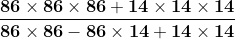Solution:

Consider x = 86 and y = 14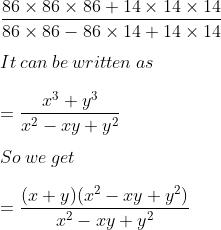= x + y

Substituting the values

= 86 + 14

= 100

### Exercise 3.2

1. If x – y = 8 and xy = 5, find x2 + y2.

Solution:

We know that

(x – y)2 = x2 + y2 – 2xy

It can be written as

x2 + y2 = (x – y)2 + 2xy

It is given that

x – y = 8 and xy = 5

Substituting the values

x2 + y2 = 82 + 2 × 5

So we get

= 64 + 10

= 74

2. If x + y = 10 and xy = 21, find 2 (x2 + y2).

Solution:

We know that

(x + y)2 = x2 + y2 + 2xy

It can be written as

x2 + y2 = (x + y)2 – 2xy

It is given that

(x + y) = 10 and xy = 21

Substituting the values

x2 + y2 = 102 – 2 × 21

By further calculation

= 100 – 42

= 58

Here

2 (x2 + y2) = 2 × 58 = 116

3. If 2a + 3b = 7 and ab = 2, find 4a2 + 9b2.

Solution:

We know that

(2a + 3b)2 = 4a2 + 9b2 + 12ab

It can be written as

4a2 + 9b2 = (2a + 3b)2 – 12ab

It is given that

2a + 3b = 7

ab = 2

Substituting the values

4a2 + 9b2 = 72 – 12 × 2

By further calculation

= 49 – 24

= 25

4. If 3x – 4y = 16 and xy = 4, find the value of 9x2 + 16y2.

Solution:

We know that

(3x – 4y)2 = 9x2 + 16y2 – 24xy

It can be written as

9x2 + 16y2 = (3x – 4y)2 + 24xy

It is given that

3x – 4y = 16 and xy = 4

Substituting the values

9x2 + 16y2 = 162 + 24 × 4

By further calculation

= 256 + 96

= 352

5. If x + y = 8 and x – y = 2, find the value of 2x2 + 2y2.

Solution:

We know that

2 (x2 + y2) = (x + y)2 + (x – y)2

It is given that

x + y = 8 and x – y = 2

Substituting the values

2x2 + 2y2 = 82 + 22

By further calculation

= 64 + 4

= 68

6. If a2 + b2 = 13 and ab = 6, find

(i) a + b

(ii) a – b

Solution:

(i) We know that

(a + b)2 = a2 + b2 + 2ab

Substituting the values

= 13 + 2 × 6

So we get

= 13 + 12

= 25

Here

a + b = ± √25 = ± 5

(ii) We know that

(a – b)2 = a2 + b2 – 2ab

Substituting the values

= 13 – 2 × 6

So we get

= 13 – 12

= 1

Here

a – b = ± √1 = ± 1

7. If a + b = 4 and ab = -12, find

(i) a – b

(ii) a2 – b2.

Solution:

(i) We know that

(a – b)2 = a2 + b2 – 2ab

It can be written as

(a – b)2 = a2 + b2 + 2ab – 4ab

(a – b)2 = (a + b)2 – 4ab

It is given that

a + b = 4 and ab = – 12

Substituting the values

(a – b)2 = 42 – 4 (-12)

By further calculation

(a – b)2 = 16 + 48 = 64

So we get

(a – b) = ± √64 = ± 8

(ii) We know that

a2 – b2 = (a + b) (a – b)

Substituting the values

a2 – b2 = 4 × ±8

a2 – b2 = ± 32

8. If p – q = 9 and pq = 36, evaluate

(i) p + q

(ii) p2 – q2.

Solution:

(i) We know that

(p + q)2 = p2 + q2 + 2pq

It can be written as

(p + q)2 = p2 + q2 – 2pq + 4pq

(p + q)2 = (p – q)2 + 4pq

It is given that

p – q = 9 and pq = 36

Substituting the values

(p + q)2 = 92 + 4 × 36

By further calculation

(p + q)2 = 81 + 144 = 225

So we get

p + q = ± √225 = ± 15

(ii) We know that

p2 – q2 = (p – q) (p + q)

Substituting the values

p2 – q2 = 9 ×±15

p2 – q2 = ± 135

9. If x + y = 6 and x – y = 4, find

(i) x2 + y2

(ii) xy

Solution:

We know that

(x + y)2 – (x – y)2 = 4xy

Substituting the values

62 – 42 = 4xy

By further calculation

36 – 16 = 4xy

20 = 4xy

4xy = 20

So we get

xy = 20/4 = 5

(i) x2 + y2 = (x + y)2 – 2xy

Substituting the values

= 62 – 2 × 5

By further calculation

= 36 – 10

= 26

(ii) xy = 5

10. If x – 3 = 1/x, find the value of x2 + 1/x2.

Solution:

It is given that

x – 3 = 1/x

We can write it as

x – 1/x = 3

Here

(x – 1/x)2 = x2 + 1/x2 – 2

So we get

x2 + 1/x2 = (x – 1/x)2 + 2

Substituting the values

x2 + 1/x2 = 32 + 2

By further calculation

= 9 + 2

= 11

11. If x + y = 8 and xy = 3 ¾, find the values of

(i) x – y

(ii) 3 (x2 + y2)

(iii) 5 (x2 + y2) + 4 (x – y).

Solution:

(i) We know that

(x – y)2 = x2 + y2 – 2xy

It can be written as

(x – y)2 = x2 + y2 + 2xy – 4xy

(x – y)2 = (x + y)2 – 4xy

It is given that

x + y = 8 and xy = 3 ¾ = 15/4

Substituting the values

(x – y)2 = 82 – 4 × 15/4

So we get

(x – y)2 = 65 – 15 = 49

x – y = ± √49 = ± 7

(ii) We know that

(x + y)2 = x2 + y2 + 2xy

We can write it as

x2 + y2 = (x + y)2 – 2xy

It is given that

x + y = 8 and xy = 3 ¾ = 15/4

Substituting the values

x2 + y2 = 82 – 2 × 15/4

So we get

x2 + y2 = 64 – 15/2

Taking LCM

x2 + y2 = (128 – 15)/ 2 = 113/2

We get

3 (x2 + y2) = 3 × 113/2 = 339/2 = 169 ½

(iii) We know that

5 (x2 + y2) + 4 (x – y) = 5 × 113/2 + 4 × ± 7

By further calculation

= 565/2 ± 28

We can write it as

= 565/2 + 28 or 565/2 – 28

= 621/2 or 509/2

It can be written as

= 310 ½ or 254 ½

12. If x2 + y2 = 34 and xy = 10 ½, find the value of 2 (x + y)2 + (x – y)2.

Solution:

It is given that

x2 + y2 = 34 and xy = 10 ½ = 21/2

We know that

(x + y)2 = x2 + y2 + 2xy

Substituting the values

(x + y)2 = 34 + 2 (21/2)

So we get

(x + y)2 = 55 ….. (1)

We know that

(x – y)2 = x2 + y2 – 2xy

Substituting the values

(x – y)2 = 34 – 2 (21/2)

So we get

(x – y)2 = 34 – 21 = 13 ….. (2)

Using both the equations

2 (x + y)2 + (x – y)2 = 2 × 55 + 13 = 123

13. If a – b = 3 and ab = 4, find a3 – b3.

Solution:

We know that

a3 – b3 = (a – b)3 + 3ab (a + b)

Substituting the values

a3 – b3 = 33 + 3 × 4 × 3

By further calculation

a3 – b3 = 27 + 36 = 63

14. If 2a – 3b = 3 and ab = 2, find the value of 8a3 – 27b3.

Solution:

We know that

8a3 – 27b3 = (2a)3 – (3b)3

According to the formula

= (2a – 3b)3 + 3 × 2a × 3b (2a – 3b)

By further simplification

= (2a – 3b)3 + 18ab (2a – 3b)

Substituting the values

= 33 + 18 × 2 × 3

By further calculation

= 27 + 108

= 135

15. If x + 1/x = 4, find the values of

(i) x2 + 1/x2

(ii) x4 + 1/x4

(iii) x3 + 1/x3

(iv) x – 1/x.

Solution:

(i) We know that

(x + 1/x)2 = x2 + 1/x2 + 2

It can be written as

x2 + 1/x2 = (x + 1/x)2 – 2

Substituting the values

= 42 – 2

= 16 – 2

= 14

(ii) We know that

(x2 + 1/x2)2 = x4 + 1/x4 + 2

It can be written as

x4 + 1/x4 = (x2 + 1/x2)2 – 2

Substituting the values

= 142 – 2

= 196 – 2

= 194

(iii) We know that

x3 + 1/x3 = (x + 1/x)3 – 3x (1/x) (x + 1/x)

It can be written as

(x + 1/x)3 – 3(x + 1/x) = 43 – 3 × 4

By further calculation

= 64 – 12

= 52

(iv) We know that

(x – 1/x)2 = x2 + 1/x2 – 2

Substituting the values

= 14 – 2

= 12

So we get

x – 1/x = ± 2√3

16. If x – 1/x = 5, find the value of x4 + 1/x4.

Solution:

We know that

(x – 1/x)2 = x2 + 1/x2 – 2

It can be written as

x2 + 1/x2 = (x – 1/x)2 + 2

Substituting the values

x2 + 1/x2 = 52 + 2 = 27

Here

x4 + 1/x4 = (x2 + 1/x2)2 – 2

Substituting the values

x4 + 1/x4 = 272 – 2

So we get

= 729 – 2

= 727

17. If x – 1/x = √5, find the values of

(i) x2 + 1/x2

(ii) x + 1/x

(iii) x3 + 1/x3

Solution:

(i) x2 + 1/x2 = (x – 1/x)2 + 2

Substituting the values

= (√5)2 + 2

= 5 + 2

= 7

(ii) (x + 1/x)2 = x2 + 1/x2 + 2

Substituting the values

= 7 + 2

= 9

Here

(x + 1/x)2 = 9

So we get

(x + 1/x) = ± √9 = ± 3

(iii) x3 + 1/x3 = (x + 1/x)3 – 3x (1/x) (x + 1/x)

Substituting the values

= (± 3)3 – 3 (± 3)

By further calculation

= (± 27) – (± 9)

= ± 18

18. If x + 1/x = 6, find

(i) x – 1/x

(ii) x2 – 1/x2.

Solution:

(i) We know that

(x – 1/x)2 = x2 + 1/x2 – 2

It can be written as

(x – 1/x)2 = x2 + 1/x2 + 2 – 4

(x – 1/x)2 = (x + 1/x)2 – 4

Substituting the values

(x – 1/x)2 = 62 – 4 = 32

So we get

x – 1/x = ± √32 = ± 4√2

(ii) We know that

x2 – 1/x2 = (x – 1/x) (x + 1/x)

Substituting the values

x2 – 1/x2 = (± 4√2) (6) = ± 24 √2

19. If x + 1/x = 2, prove that x2 + 1/x2 = x3 + 1/x3 = x4 + 1/x4.

Solution:

We know that

x2 + 1/x2 = (x + 1/x) – 2

Substituting the values

x2 + 1/x2 = 22 – 2

So we get

x2 + 1/x2 = 4 – 2 = 2 …. (1)

x3 + 1/x3 = (x + 1/x)3 – 3 (x + 1/x)

Substituting the values

x3 + 1/x3 = 23 – 3 × 2

So we get

x3 + 1/x3 = 8 – 6 = 2 …… (2)

x4 + 1/x4 = (x2 + 1/x2)2 – 2

Substituting the values

x4 + 1/x4 = 22 – 2

So we get

x4 + 1/x4 = 4 – 2 = 2 …. (3)

From equation (1), (2) and (3)

x2 + 1/x2 = x3 + 1/x3 = x4 + 1/x4

Hence, it is proved.

20. If x – 2/x = 3, find the value of x3 – 8/x3.

Solution:

We know that

(x – 2/x)3 = x3 – 8/x3 – 3 (x) (2/x) (x – 2/x)

By further simplification

(x – 2/x)3 = x3 – 8/x3 – 6 (x – 2/x)

It can be written as

x3 – 8/x3 = (x – 2/x)3 + 6 (x – 2/x)

Substituting the values

x3 – 8/x3 = 33 + 6 × 3

By further calculation

x3 – 8/x3 = 27 + 18 = 45

21. If a + 2b = 5, prove that a3 + 8b3 + 30ab = 125.

Solution:

We know that

(a + 2b)3 = a3 + 8b3 + 3 (a) (2b) (a + 2b)

Substituting the values

53 = a3 + 8b3 + 6ab (5)

By further calculation

125 = a3 + 8b3 + 30ab

Therefore, a3 + 8b3 + 30ab = 125.

22. If a + 1/a = p, prove that a3 + 1/a3 = p (p2 – 3).

Solution:

We know that

a3 + 1/a3 = (a + 1/a)3 – 3a (1/a) (a + 1/a)

Substituting the values

a3 + 1/a3 = p3 – 3p

Taking p as common

a3 + 1/a3 = p (p2 – 3)

Therefore, it is proved.

23. If x2 + 1/x2 = 27, find the value of x – 1/x.

Solution:

We know that

(x – 1/x)2 = x2 + 1/x2 – 2

Substituting the values

(x – 1/x)2 = 27 – 2 = 25

So we get

x – 1/x = ± √25 = ± 5

24. If x2 + 1/x2 = 27, find the value of 3x3 + 5x – 3/x3 – 5/x.

Solution:

We know that

(x – 1/x)2 = x2 + 1/x2 – 2

Substituting the values

(x – 1/x)2 = 27 – 2 = 25

So we get

x – 1/x = ± √25 = ± 5

Here

3x3 + 5x – 3/x3 – 5/x = 3 (x3 – 1/x3) + 5 (x – 1/x)

It can be written as

= 3 [(x – 1/x)3 + 3 (x – 1/x)] + 5 (x – 1/x)

Substituting the values

= 3 [(± 5)3 + 3 (± 5)] + 5 (± 5)

By further calculation

= 3 [(± 125) + (± 15)] + (± 25)

So we get

= (± 375) + (± 45) + (± 25)

= ± 445

25. If x2 + 1/25x2 = 8 3/5, find x + 1/5x.

Solution:

We know that

(x + 1/5x)2 = x2 + 1/25x2 + 2x (1/5x)

It can be written as

(x + 1/5x)2 = x2 + 1/25x2 + 2/5

Substituting the values

(x + 1/5x)2 = 8 3/5 + 2/5

(x + 1/5x)2 = 43/5 + 2/5

So we get

(x + 1/5x)2 = 45/5 = 9

Here

x + 1/5x = ± √9 = ± 3

26. If x2 + 1/4x2 = 8, find x3 + 1/8x3.

Solution:

We know that

(x + 1/2x)2 = x2 + (1/2x)2 + 2x (1/2x)

It can be written as

(x + 1/2x)2 = x2 + 1/4x2 + 1

Substituting the values

(x + 1/2x)2 = 8 + 1 = 9

So we get

x + 1/2x = ± √9 = ± 3

Here

x3 + 1/8x3 = x3 + (1/2x)3

We know that

x3 + 1/8x3 = (x + 1/2x)3 – 3x (1/2x) (x + 1/2x)

Substituting the values

x3 + 1/8x3 = (± 3)3 – 3/2 (± 3)

By further calculation

x3 + 1/8x3 = ± (27 – 9/2)

Taking LCM

x3 + 1/8x3 = ± (54 – 9)/ 2

x3 + 1/8x3 = ± 45/2 = ± 22 ½

Therefore, x3 + 1/8x3 = ± 22 ½.

27. If a2 – 3a + 1 = 0, find

(i) a2 + 1/a2

(ii) a3 + 1/a3.

Solution:

It is given that

a2 – 3a + 1 = 0

By dividing each term by a

a + 1/a = 3

(i) We know that

(a + 1/a)2 = a2 + 1/a2 + 2

It can be written as

a2 + 1/a2 = (a + 1/a)2 – 2

Substituting the values

= 32 – 2

= 9 – 2

= 7

(ii) We know that

(a + 1/a)3 = a3 + 1/a3 + 3 (a + 1/a)

It can be written as

a3 + 1/a3 = (a + 1/a)3 – 3 (a + 1/a)

Substituting the values

= 33 – 3 (3)

= 27 – 9

= 18

28. If a = 1/ (a – 5), find

(i) a – 1/a

(ii) a + 1/a

(iii) a2 – 1/a2.

Solution:

It is given that

a = 1/ (a – 5)

We can write it as

a2 – 5a – 1 = 0

Now divide each term by a

a – 5 – 1/a = 0

So we get

a – 1/a = 5

(i) a – 1/a = 5

(ii) We know that

(a + 1/a)2 = (a – 1/a)2 + 4

Substituting the values

(a + 1/a)2 = 52 + 4

So we get

(a + 1/a)2 = 25 + 4 = 29

a + 1/a = ± √29

(ii) We know that

a2 – 1/a2 = (a + 1/a) (a – 1/a)

Substituting the values

a2 – 1/a2 = ± √29 × 5

a2 – 1/a2 = ± 5√29

29. If (x + 1/x)2 = 3, find x3 + 1/x3.

Solution:

It is given that

(x + 1/x)2 = 3

(x + 1/x) = ± √3

We know that

x3 + 1/x3 = (x + 1/x)3 – 3 (x + 1/x)

Substituting the values

x3 + 1/x3 = (± √3)3 – 3 (± √3)

By further calculation

x3 + 1/x3 = (± 3√3) – (± 3√3) = 0

30. If x = 5 – 2√6, find the value of √x + 1/√x.

Solution:

It is given that

x = 5 – 2√6

We can write it as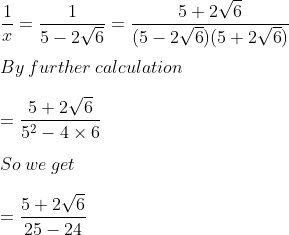= 5 + 2√6

Here

x + 1/x = 5 – 2√6 + 5 + 2√6 = 10

So we get

(√x + 1/√x)2 = x + 1/x + 2

Substituting the values

= 10 + 2

= 12

31. If a + b + c = 12 and ab + bc + ca = 22, find a2 + b2 + c2.

Solution:

We know that

(a + b + c)2 = a2 + b2 + c2 + 2(ab + bc + ca)

We can write it as

a2 + b2 + c2 = (a + b + c)2 – 2 (ab + bc + ca)

Substituting the values

a2 + b2 + c2 = 122 – 2 (22)

By further calculation

a2 + b2 + c2 = 144 – 44 = 100

32. If a + b + c = 12 and a2 + b2 + c2 = 100, find ab + bc + ca.

Solution:

We know that

(a + b + c)2 = a2 + b2 + c2 + 2ab + 2bc + 2ca

It can be written as

2ab + 2bc + 2ca = (a + b + c)2 – (a2 + b2 + c2)

Taking out 2 as common

2 (ab + bc + ca) = 122 – 100 = 144 – 100 = 44

By further calculation

ab + bc + ca = 44/2 = 22

33. If a2 + b2 + c2 = 125 and ab + bc + ca = 50, find a + b + c.

Solution:

We know that

(a + b + c)2 = a2 + b2 + c2 + 2(ab + bc + ca)

Substituting the values

(a + b + c)2 = 125 + 2 (50)

By further calculation

(a + b + c)2 = 125 + 100 = 225

So we get

a + b + c = ± √225 = ± 15

34. If a + b – c = 5 and a2 + b2 + c2 = 29, find the value of ab – bc – ca.

Solution:

It is given that

a + b – c = 5

By squaring on both sides

(a + b – c)2 = 52

Expanding using formula

a2 + b2 + c2 + 2ab – 2bc – 2ca = 25

Substituting the values and taking 2 as common

29 + 2 (ab – bc – ca) = 25

By further calculation

2 (ab – bc – ca) = 25 – 29 = – 4

So we get

ab – bc – ca = – 4/2 = – 2

Therefore, ab – bc – ca = – 2.

35. If a – b = 7 and a2 + b2 = 85, then find the value of a3 – b3.

Solution:

We know that

(a – b)2 = a2 + b2 – 2ab

Substituting the values

72 = 85 – 2ab

By further calculation

49 = 85 – 2ab

So we get

2ab = 85 – 49 = 36

Dividing by 2

ab = 36/2 = 18

Here

a3 – b3 = (a – b) (a2 + b2 + ab)

Substituting the values

a3 – b3 = 7 (85 + 18)

By further calculation

a3 – b3 = 7 × 103

So we get

a3 – b3 = 721

36. If the number x is 3 less than the number y and the sum of the squares of x and y is 29, find the product of x and y.

Solution:

It is given that

x = y – 3 and x2 + y2 = 29

It can be written as

x – y = – 3

By squaring on both sides

(x – y)2 = (-3)2

Expanding using formula

x2 + y2 – 2xy = 9

Substituting the values

29 – 2xy = 9

By further calculation

-2xy = 9 – 29 = – 20

Dividing by 2

xy = – 20/-2 = 10

So we get

xy = 10

37. If the sum and the product of two numbers are 8 and 15 respectively, find the sum of their cubes.

Solution:

Consider x and y as the two numbers

x + y = 8 and xy = 15

By cubing on both sides

(x + y)3 = 83

Expanding using formula

x3 + y3 + 3xy (x + y) = 512

Substituting the values

x3 + y3 + 3 × 15 × 8 = 512

By further calculation

x3 + y3 + 360 = 512

So we get

x3 + y3 = 512 – 360 = 152

Chapter Test

1. Find the expansions of the following :
(i) (2x + 3y + 5) (2x + 3y – 5)
(ii) (6 – 4a -7b)2
(iii) (7 – 3xy)3
(iv) (x + y + 2)3

Solution:

(i) (2x + 3y + 5) (2x + 3y – 5)

Let us simplify the expression, we get

(2x + 3y + 5) (2x + 3y – 5) = [(2x + 3y) + 5] [(2x – 3y) – 5]

By using the formula, (a)2 – (b)2 = [(a + b) (a – b)]
= (2x + 3y)2 – (5)2

= (2x)2 + (3y) 2 + 2 × 2x × 3y – 5 × 5

= 4x2 + 9y2 + 12xy – 25

(ii) (6 – 4a – 7 b)2

Let us simplify the expression, we get

(6 – 4a – 7 b)2 = [ 6 + (- 4a) + (-7b)]2

= (6)2 + (- 4a)2 + (- 7b)2 + 2 (6) (- 4a) + 2 (- 4a) (-7b) + 2 (-7b) (6)

= 36 + 16a2 + 49b2 – 48a + 56ab – 84b

(iii) (7 – 3xy)3

Let us simplify the expression

By using the formula, we get

(7 – 3xy)3 = (7)3 – (3xy)3 – 3 (7) (3xy) (7 – 3xy)

= 343 – 27x3y3 – 63xy (7 – 3xy)

= 343 – 27x3y3 – 441xy + 189x2y2

(iv) (x + y + 2)3

Let us simplify the expression

By using the formula, we get

(x + y + 2 )3 = [(x + y) + 2]3

= (x + y)3 + (2)3 + 3 (x + y) (2) (x + y + 2)

= x3 + y3 + 3x2y + 3xy2 + 8 + 6 (x + y) [(x + y) + 2]
= x3 + y3 + 3x2y + 3xy2 + 8 + 6 (x + y)2 +12(x + y)

= x3 + y3 + 3x2y + 3xy2 + 8 + 6 (x2 + y2 + 2xy) + 12x + 12y = x3 + y3 + 3x2y + 3xy2 + 8 + 6x2 + 6y2 + 12xy + 12x + 12y

= x3 + y3 + 3x2y + 3xy2 + 8 + 6x2 + 6y2 + 12x + 12y + 12xy

2. Simplify: (x – 2) (x + 2) (x2 + 4) (x4 + 16)
Solution:

Let us simplify the expression, we get

(x – 2) (x + 2) (x4 + 4) (x4 +16) = (x2 – 4) (x4 + 4) (x4 + 16)

= [(x2)2 – (4)2] (x4 + 16)

= (x4 – 16) (x4 + 16)

= (x4)2 – (16)2

= x8 – 256

3. Evaluate 1002 × 998 by using a special product.
Solution:

Let us simplify the expression, we get

1002 × 998 = (1000 + 2) (1000 – 2)

= (1000)2 – (2)2

= 1000000 – 4

= 999996

4. If a + 2b + 3c = 0, Prove that a3 + 8b3 + 27c3 = 18 abc
Solution:

Given:

a + 2b + 3c = 0, a + 2b = – 3c

Let us cube on both the sides, we get

(a + 2b)3 = (-3c)3

a3 + (2b)3 + 3(a) (2b) (a + 2b) = -27c3

a3 + 8b3 + 6ab (– 3c) = – 27c3

a3 + 8b3 – 18abc = -27c3

a3 + 8b3 + 27c3 = 18abc

Hence proved.

5. If 2x = 3y – 5, then find the value of 8x3 – 27y3 + 90xy + 125.
Solution:

Given:

2x = 3y – 5

2x – 3y = -5

Now, let us cube on both sides, we get

(2x – 3y)3 = (-5)3

(2x)3 – (3y)3 – 3 × 2x × 3y (2x – 3y) = -125

8x3 – 27y3 – 18xy (2x – 3y) = -125

Now, substitute the value of 2x – 3y = -5

8x3 – 27y3 – 18xy (-5) = -125

8x3 – 27y3 + 90xy = -125

8x3 – 27y3 + 90xy + 125 = 0

6. If a2 – 1/a2 = 5, evaluate a4 + 1/a4

Solution:

It is given that,

a2 – 1/a2 = 5

So,

By using the formula, (a + b)2

[a2 – 1/a2]2 = a4 + 1/a4 – 2

[a2 – 1/a2]2 + 2 = a4 + 1/a4

Substitute the value of a2 – 1/a2 = 5, we get

52 + 2 = a4 + 1/a4

a4 + 1/a4 = 25 + 2

= 27

7. If a + 1/a = p and a – 1/a = q, Find the relation between p and q.

Solution:

It is given that,

a + 1/a = p and a – 1/a = q

so,

(a + 1/a)2 – (a – 1/a)2 = 4(a) (1/a)

= 4

By substituting the values, we get

p2 – q2 = 4

Hence the relation between p and q is that p2 – q2 = 4.

8. If (a2 + 1)/a = 4, find the value of 2a3 + 2/a3

Solution:

It is given that,

(a2 + 1)/a = 4

a2/a + 1/a = 4

a + 1/a = 4

So by multiplying the expression by 2a, we get

2a3 + 2/a3 = 2[a3 + 1/a3]

= 2 [(a + 1/a)3 – 3 (a) (1/a) (a + 1/a)]

= 2 [(4)3 – 3(4)]

= 2 [64 – 12]

= 2 (52)

= 104

9. If x = 1/(4 – x), find the value of

(i) x + 1/x

(ii) x3 + 1/x3

(iii) x6 + 1/x6

Solution:

It is given that,

x = 1/(4 – x)

So,

(i) x(4 – x) = 1

4x – x2 = 1

Now let us divide both sides by x, we get

4 – x = 1/x

4 = 1/x + x

1/x + x = 4

1/x + x = 4

(ii) x3 + 1/x3 = (x + 1/x)2 – 3(x + 1/x)

By substituting the values, we get

= (4)3 – 3(4)

= 64 – 12

= 52

(iii) x6 + 1/x6 = (x3 + 1/x3)2 – 2

= (52)2 – 2

= 2704 – 2

= 2702

10. If x – 1/x = 3 + 2√2, find the value of ¼ (x3 – 1/x3)

Solution:

It is given that,

x – 1/x = 3 + 2√2

So,

x3 – 1/x3 = (x – 1/x)3 + 3(x – 1/x)

= (3 + 2√2)3 + 3(3 + 2√2)

By using the formula, (a+b)3 = a3 + b3 + 3ab (a + b)

= (3)3 + (2√2)3 + 3 (3) (2√2) (3 + 2√2) + 3(3 + 2√2)

= 27 + 16√2 + 54√2 + 72 + 9 + 6√2

= 108 + 76√2

Hence,

¼ (x3 – 1/x3) = ¼ (108 + 76√2)

= 27 + 19√2

11. If x + 1/x = 3 1/3, find the value of x3 – 1/x3

Solution:

It is given that,

x + 1/x = 3 1/3

we know that,

(x – 1/x)2 = x2 + 1/x2 – 2

= x2 + 1/x2 + 2 – 4

= (x + 1/x)2 – 4

But x + 1/x = 3 1/3 = 10/3

So,

(x – 1/x)2 = (10/3)2 – 4

= 100/9 – 4

= (100 – 36)/9

= 64/9

x – 1/x = √(64/9)

= 8/3

Now,

x3 – 1/x3 = (x – 1/x)3 + 3 (x) (1/x) (x – 1/x)

= (8/3)3 + 3 (8/3)

= ((512/27) + 8)

= 728/27

= 26 26/27

12. If x = 2 – √3, then find the value of x3 – 1/x3

Solution:

It is given that,

x = 2 – √3

so,

1/x = 1/(2 – √3)

By rationalizing the denominator, we get

= [1(2 + √3)] / [(2 – √3) (2 + √3)]

= [(2 + √3)] / [(22) – (√3)2]

= [(2 + √3)] / [4 – 3]

= 2 + √3

Now,

x – 1/x = 2 – √3 – 2 – √3

= – 2√3

Let us cube on both sides, we get

(x – 1/x)3 = (-2√3)3

x3 – 1/x3 – 3 (x) (1/x) (x – 1/x) = 24√3

x3 – 1/x3 – 3(-2√3) = -24√3

x3 – 1/x3 + 6√3 = -24√3

x3 – 1/x3 = -24√3 – 6√3

= -30√3

Hence,

x3 – 1/x3 = -30√3

13. If the sum of two numbers is 11 and sum of their cubes is 737, find the sum of their squares.
Solution:

Let us consider x and y be two numbers

Then,

x + y = 11

x3 + y3 = 735 and x2 + y2 =?

Now,

x + y = 11

Let us cube on both the sides,

(x + y)3 = (11)3

x3 + y3 + 3xy (x + y) = 1331

737 + 3x × 11 = 1331

33xy = 1331 – 737

= 594

xy = 594/33

xy = 8

We know that, x + y = 11

By squaring on both sides, we get

(x + y)2 = (11)2

x2 + y2 + 2xy = 121 2 x2 + y2 + 2 × 18 = 121

x2 + y2 + 36 = 121

x2 + y2 = 121 – 36

= 85

Hence sum of the squares = 85

14. If a – b = 7 and a3 – b3 = 133, find:
(i) ab
(ii) a2 + b2
Solution:

It is given that,

a – b = 7

let us cube on both sides, we get

(i) (a – b)3 = (7)3

a3 + b3 – 3ab (a – b) = 343

133 – 3ab × 7 = 343

133 – 21ab = 343

– 21ab = 343 – 133 21ab

= 210

ab = -210/21

ab = -10

(ii) a2 + b2

Again a – b = 7

Let us square on both sides, we get

(a – b)2 = (7)2

a2 + b2 – 2ab = 49

a2 + b2 – 2 × (- 10) = 49

a2 + b2 + 20 = 49

a2 + b2 = 49 – 20

= 29

Hence, a2 + b2 = 29

15. Find the coefficient of x2 expansion of (x2 + x + 1)2 + (x2 – x + 1)2
Solution:

Given:

The expression, (x2 + x + 1)2 + (x2 – x + 1)2

(x2 + x + 1)2 + (x2 – x + 1)2 = [((x2 + 1) + x)2 + [(x2 + 1) – x)2]
= (x2 + 1)2 + x2 + 2 (x2 + 1) (x) + (x2 + 1)2 + x2 – 2 (x2 + 1) (x)

= (x2)2 + (1)2 + 2 × x2 × 1 + x2 + (x2)2 + 1 + 2 × x2 + 1 + x2

= x4 + 1 + 2x2 + x2 + x4 + 1 + 2x2 + x2

= 2x4 + 6x2 + 2

∴ Co-efficient of x2 is 6.